Research papers and code for "Yu Ma":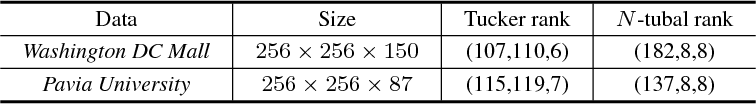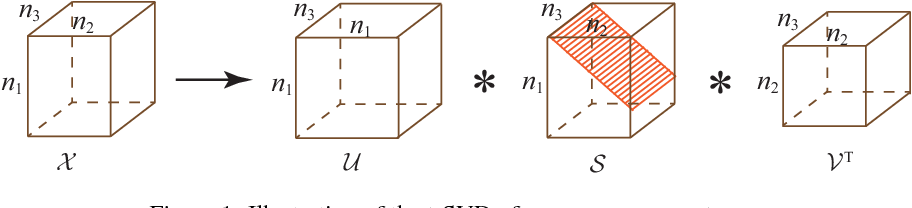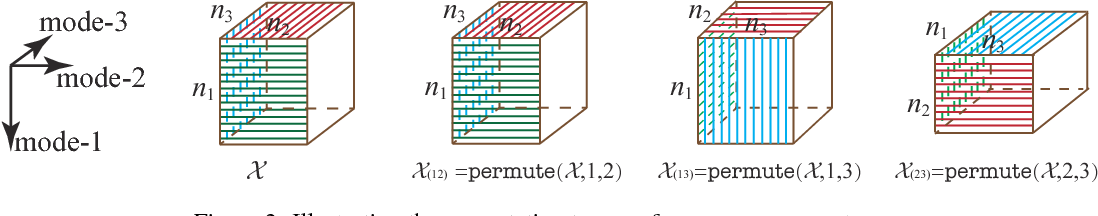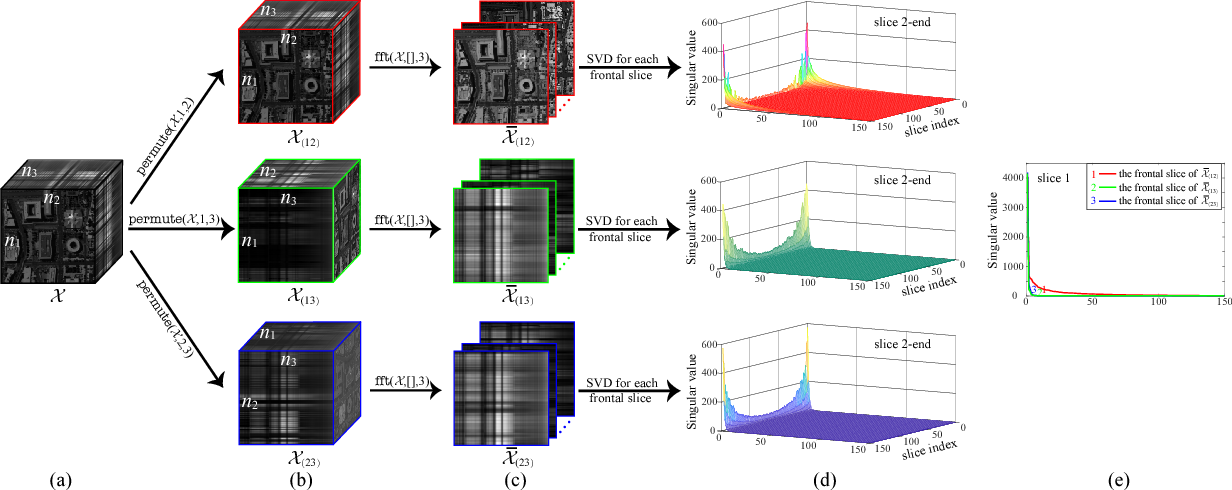As low-rank modeling has achieved great success in tensor recovery, many research efforts devote to defining the tensor rank. Among them, the recent popular tensor tubal rank, defined based on the tensor singular value decomposition (t-SVD), obtains promising results. However, the framework of the t-SVD and the tensor tubal rank are applicable only to three-way tensors and lack of flexibility to handle different correlations along different modes. To tackle these two issues, we define a new tensor unfolding operator, named mode-$k_1k_2$ tensor unfolding, as the process of lexicographically stacking the mode-$k_1k_2$ slices of an $N$-way tensor into a three-way tensor, which is a three-way extension of the well-known mode-$k$ tensor matricization. Based on it, we define a novel tensor rank, the tensor $N$-tubal rank, as a vector whose elements contain the tubal rank of all mode-$k_1k_2$ unfolding tensors, to depict the correlations along different modes. To efficiently minimize the proposed $N$-tubal rank, we establish its convex relaxation: the weighted sum of tensor nuclear norm (WSTNN). Then, we apply WSTNN to low-rank tensor completion (LRTC) and tensor robust principal component analysis (TRPCA). The corresponding WSTNN-based LRTC and TRPCA models are proposed, and two efficient alternating direction method of multipliers (ADMM)-based algorithms are developed to solve the proposed models. Numerical experiments demonstrate that the proposed models significantly outperform the compared ones.

Click to Read Paper and Get Code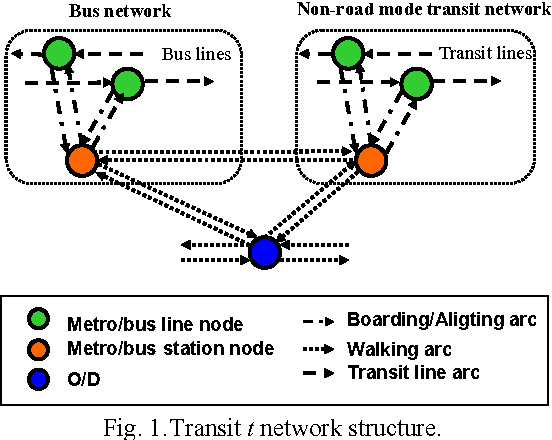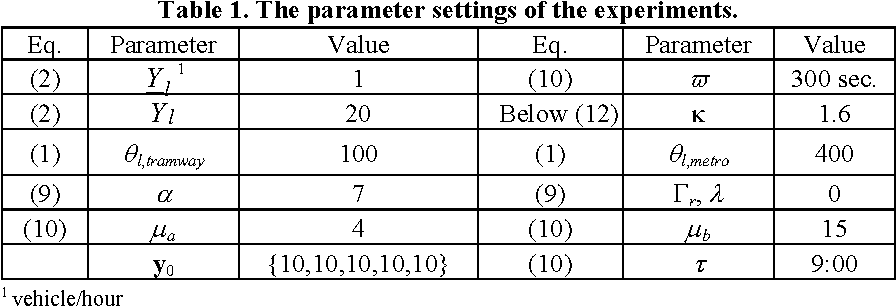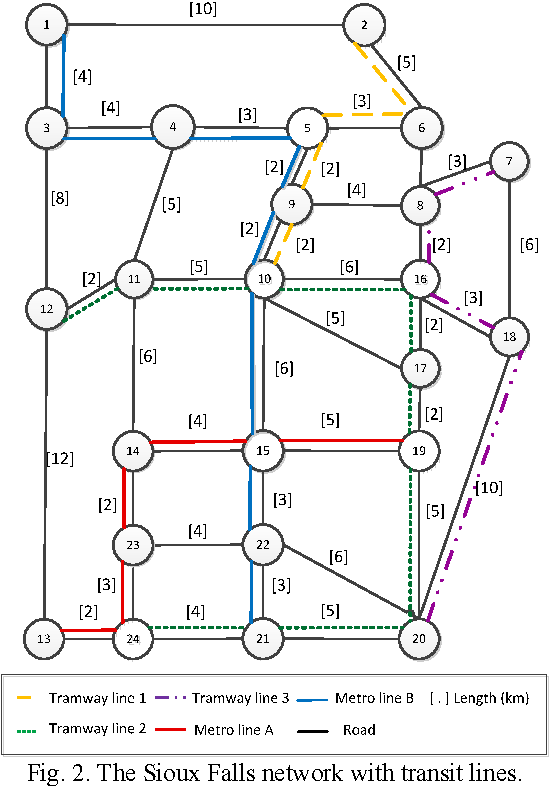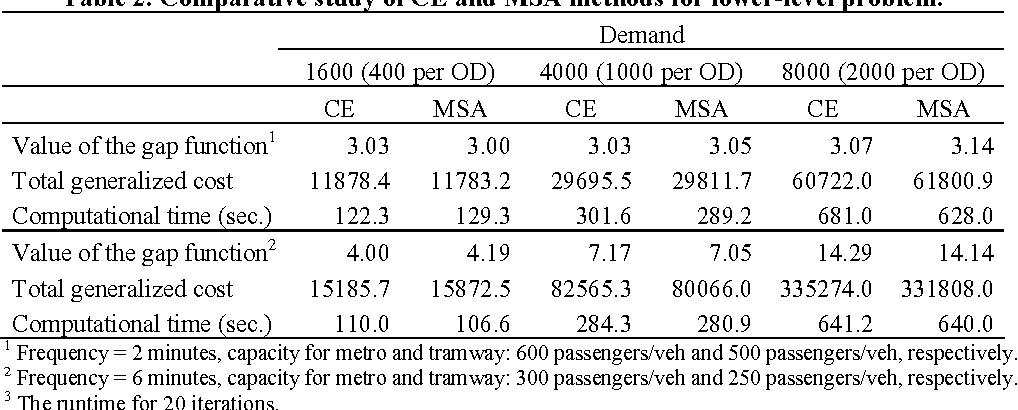This paper proposes a hybrid multiagent learning algorithm for solving the dynamic simulation-based bilevel network design problem. The objective is to determine the op-timal frequency of a multimodal transit network, which minimizes total users' travel cost and operation cost of transit lines. The problem is formulated as a bilevel programming problem with equilibrium constraints describing non-cooperative Nash equilibrium in a dynamic simulation-based transit assignment context. A hybrid algorithm combing the cross entropy multiagent learning algorithm and Hooke-Jeeves algorithm is proposed. Computational results are provided on the Sioux Falls network to illustrate the perform-ance of the proposed algorithm.

Click to Read Paper and Get Code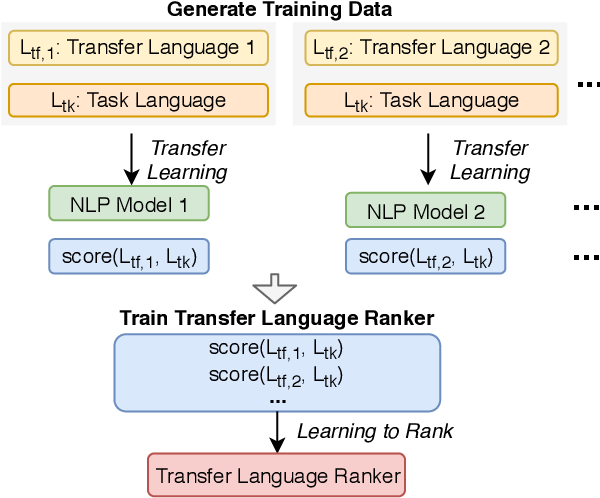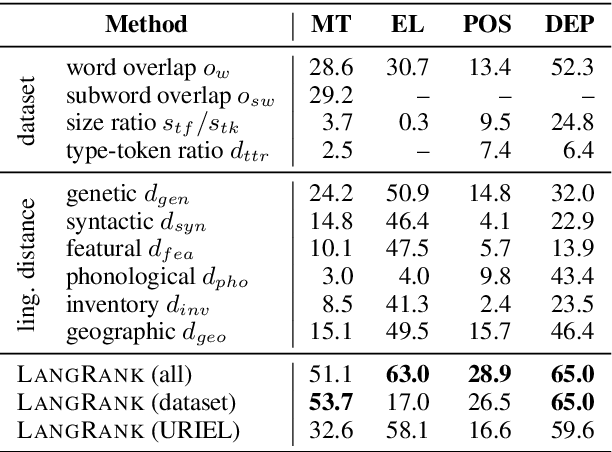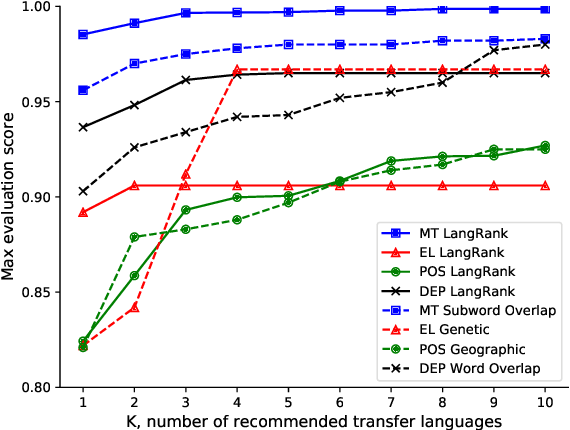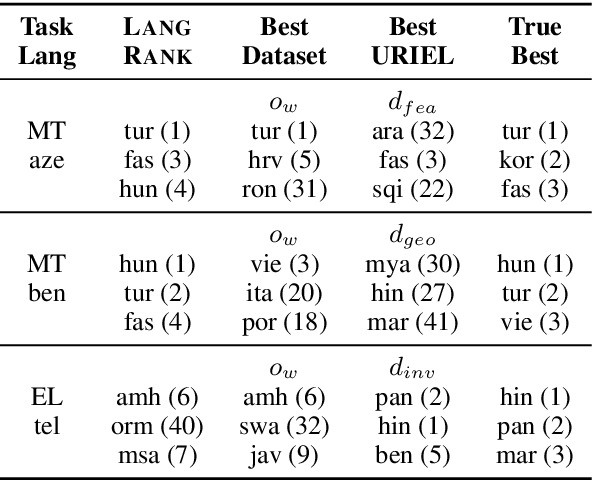Cross-lingual transfer, where a high-resource transfer language is used to improve the accuracy of a low-resource task language, is now an invaluable tool for improving performance of natural language processing (NLP) on low-resource languages. However, given a particular task language, it is not clear which language to transfer from, and the standard strategy is to select languages based on ad hoc criteria, usually the intuition of the experimenter. Since a large number of features contribute to the success of cross-lingual transfer (including phylogenetic similarity, typological properties, lexical overlap, or size of available data), even the most enlightened experimenter rarely considers all these factors for the particular task at hand. In this paper, we consider this task of automatically selecting optimal transfer languages as a ranking problem, and build models that consider the aforementioned features to perform this prediction. In experiments on representative NLP tasks, we demonstrate that our model predicts good transfer languages much better than ad hoc baselines considering single features in isolation, and glean insights on what features are most informative for each different NLP tasks, which may inform future ad hoc selection even without use of our method. Code, data, and pre-trained models are available at https://github.com/neulab/langrank

* Proceedings of ACL 2019
Click to Read Paper and Get Code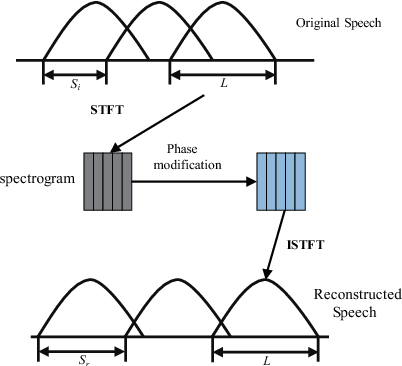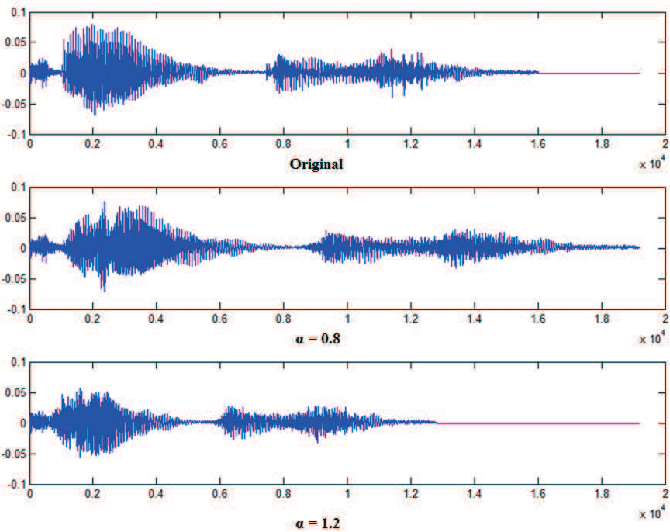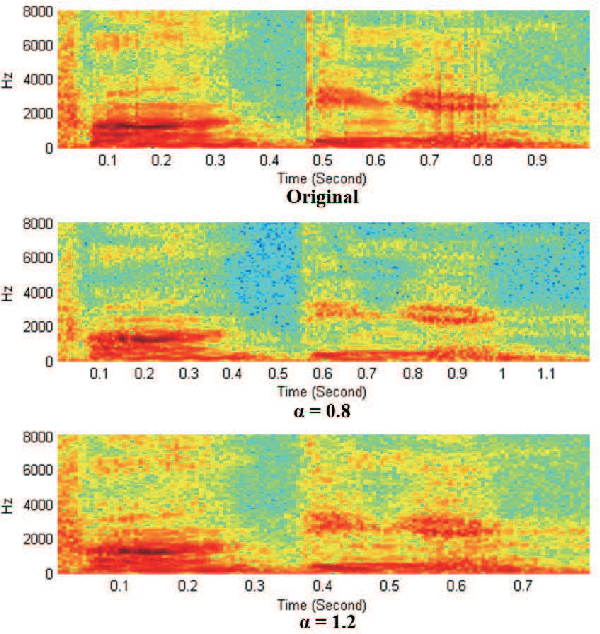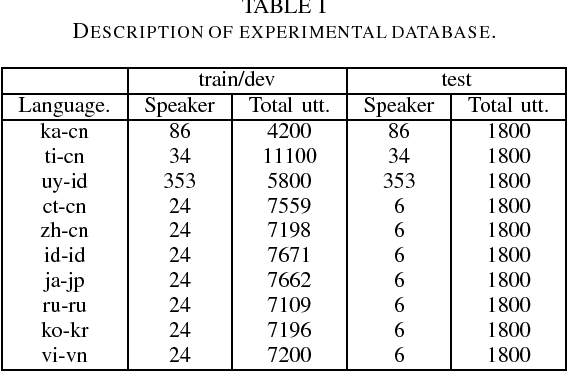In this paper we proposed an end-to-end short utterances speech language identification(SLD) approach based on a Long Short Term Memory (LSTM) neural network which is special suitable for SLD application in intelligent vehicles. Features used for LSTM learning are generated by a transfer learning method. Bottle-neck features of a deep neural network (DNN) which are trained for mandarin acoustic-phonetic classification are used for LSTM training. In order to improve the SLD accuracy of short utterances a phase vocoder based time-scale modification(TSM) method is used to reduce and increase speech rated of the test utterance. By splicing the normal, speech rate reduced and increased utterances, we can extend length of test utterances so as to improved improved the performance of the SLD system. The experimental results on AP17-OLR database shows that the proposed methods can improve the performance of SLD, especially on short utterance with 1s and 3s durations.

* Preliminary work report
Click to Read Paper and Get Code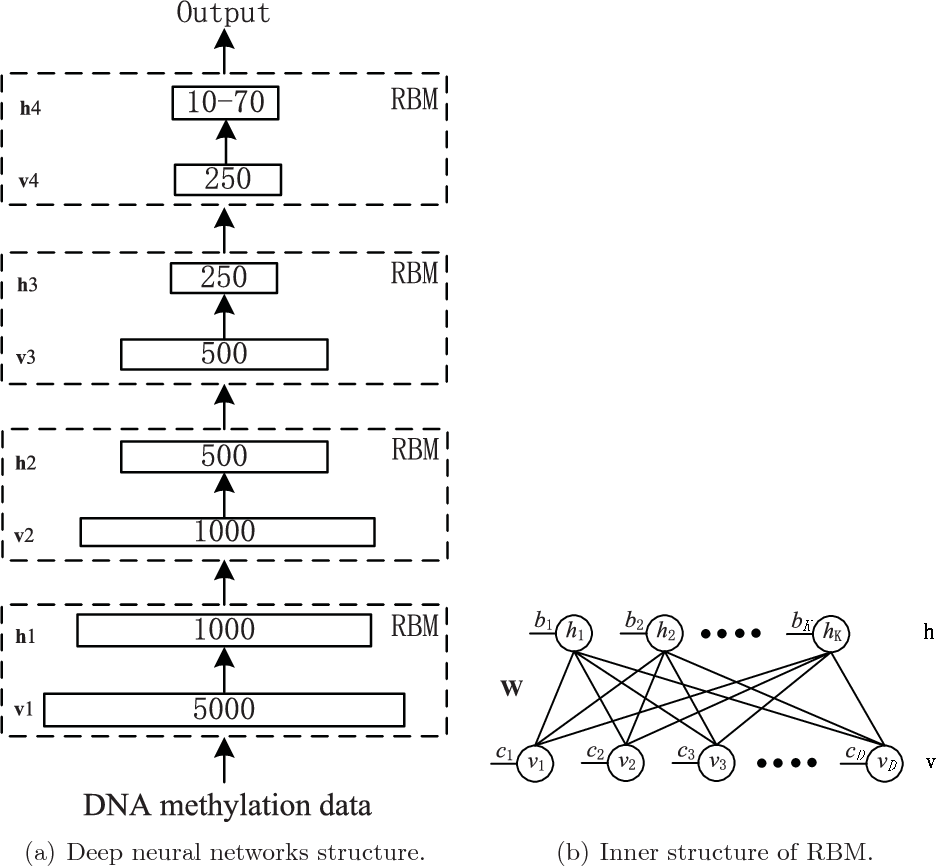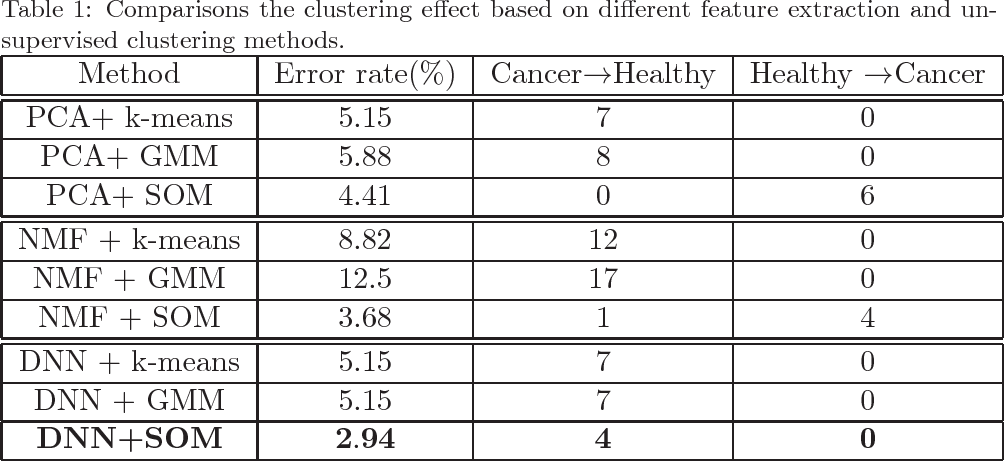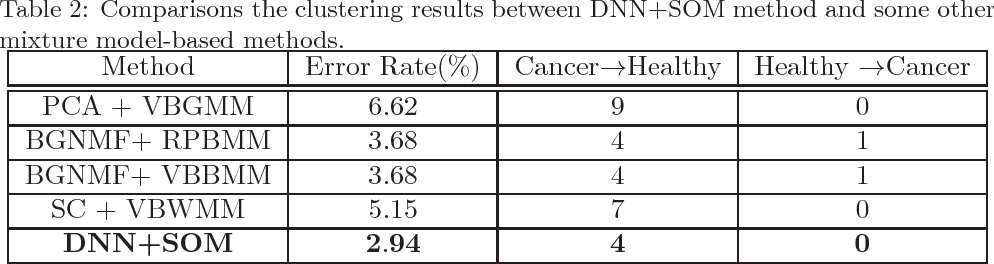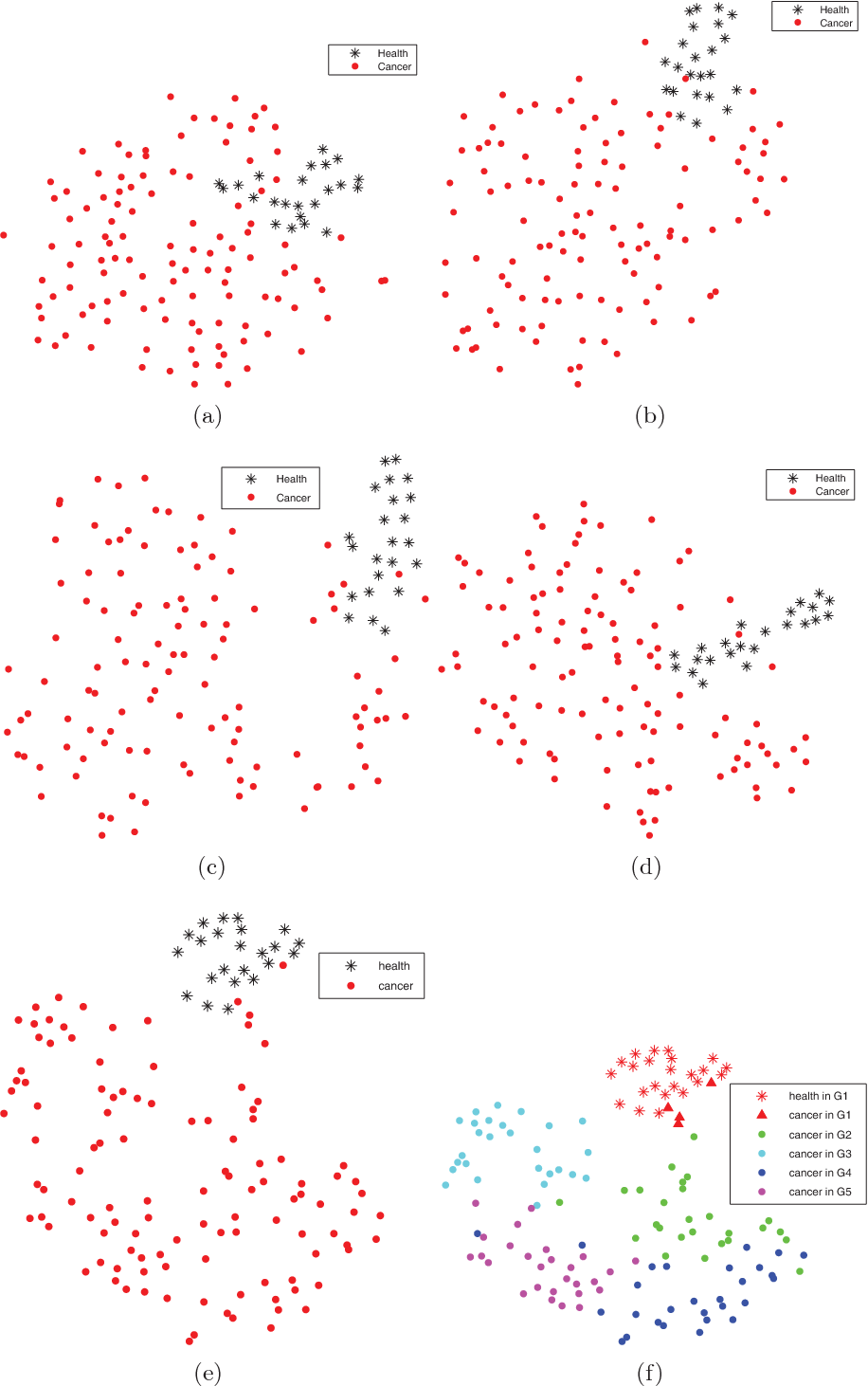Many researches demonstrated that the DNA methylation, which occurs in the context of a CpG, has strong correlation with diseases, including cancer. There is a strong interest in analyzing the DNA methylation data to find how to distinguish different subtypes of the tumor. However, the conventional statistical methods are not suitable for analyzing the highly dimensional DNA methylation data with bounded support. In order to explicitly capture the properties of the data, we design a deep neural network, which composes of several stacked binary restricted Boltzmann machines, to learn the low dimensional deep features of the DNA methylation data. Experiments show these features perform best in breast cancer DNA methylation data cluster analysis, comparing with some state-of-the-art methods.

* Techinical Report
Click to Read Paper and Get Code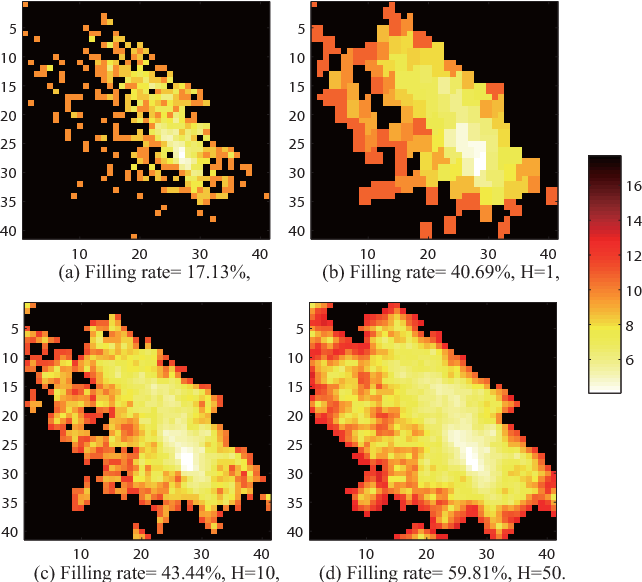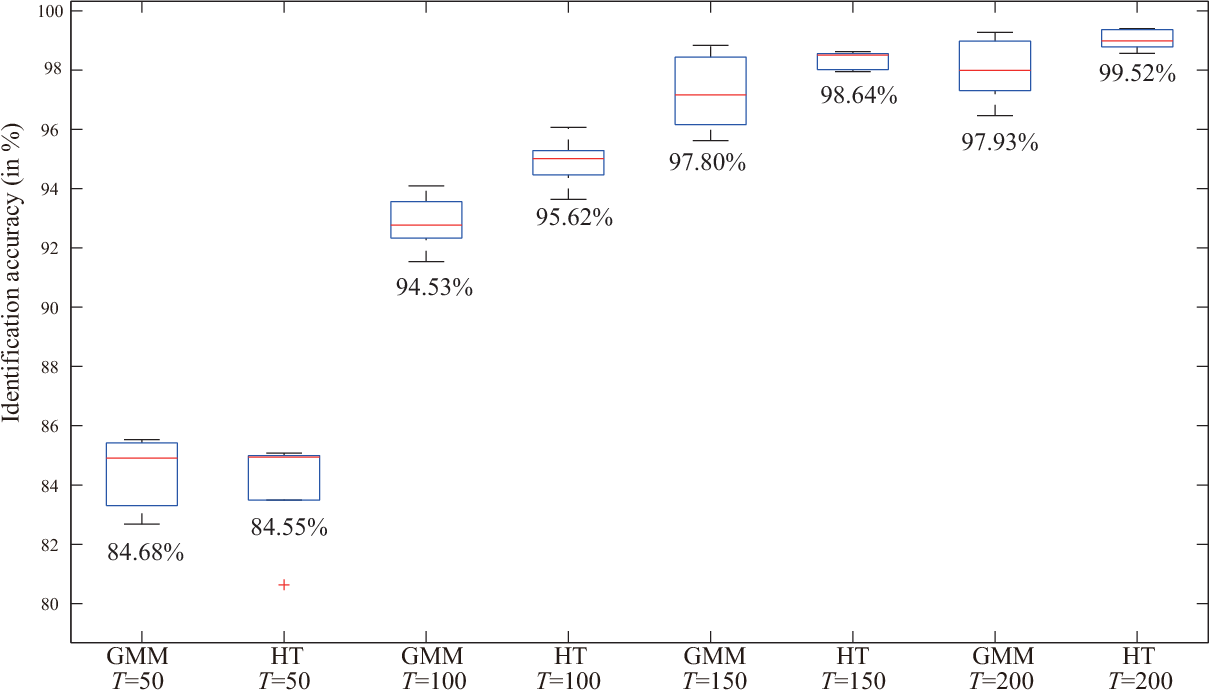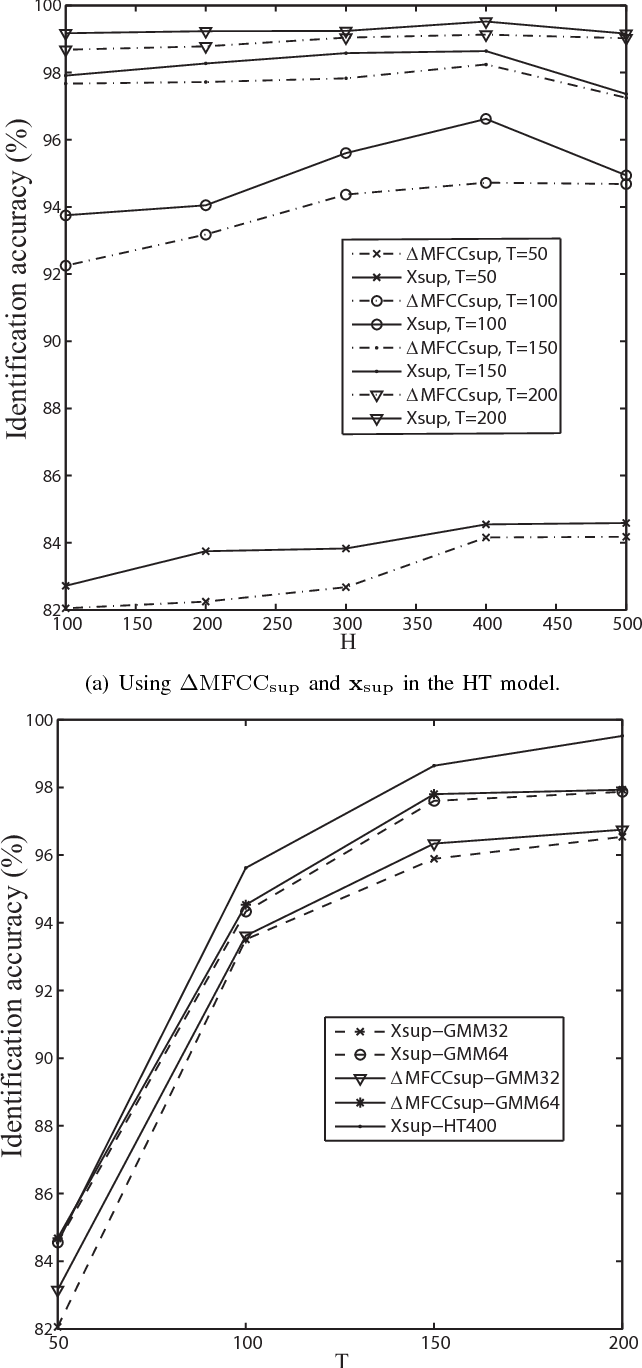A novel text-independent speaker identification (SI) method is proposed. This method uses the Mel-frequency Cepstral coefficients (MFCCs) and the dynamic information among adjacent frames as feature sets to capture speaker's characteristics. In order to utilize dynamic information, we design super-MFCCs features by cascading three neighboring MFCCs frames together. The probability density function (PDF) of these super-MFCCs features is estimated by the recently proposed histogram transform~(HT) method, which generates more training data by random transforms to realize the histogram PDF estimation and recedes the commonly occurred discontinuity problem in multivariate histograms computing. Compared to the conventional PDF estimation methods, such as Gaussian mixture models, the HT model shows promising improvement in the SI performance.

* Technical Report
Click to Read Paper and Get Code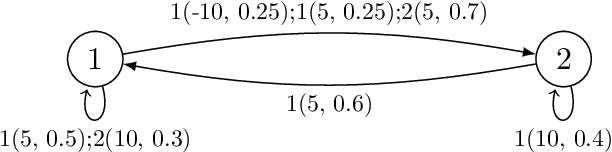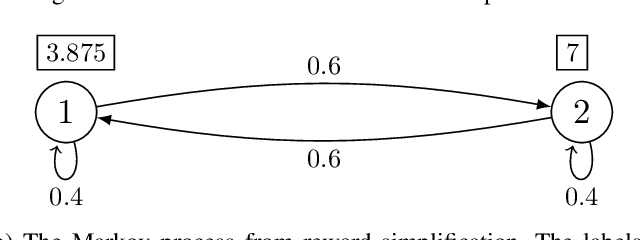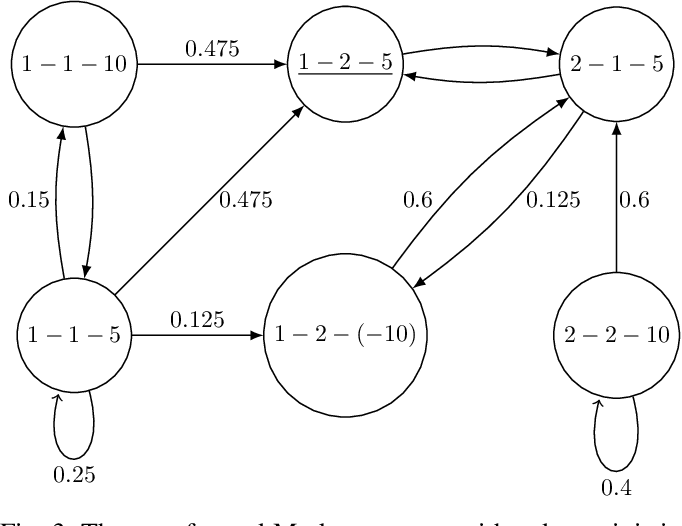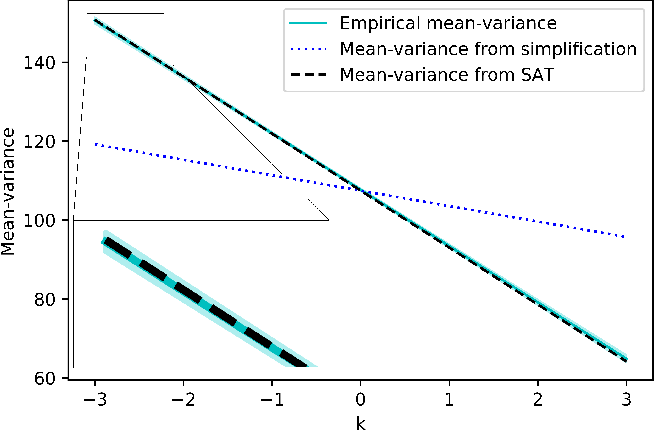Variance plays a crucial role in risk-sensitive reinforcement learning, and most risk measures can be analyzed via variance. In this paper, we consider two law-invariant risks as examples: mean-variance risk and exponential utility risk. With the aid of the state-augmentation transformation (SAT), we show that, the two risks can be estimated in Markov decision processes (MDPs) with a stochastic transition-based reward and a randomized policy. To relieve the enlarged state space, a novel definition of isotopic states is proposed for state lumping, considering the special structure of the transformed transition probability. In the numerical experiment, we illustrate state lumping in the SAT, errors from a naive reward simplification, and the validity of the SAT for the two risk estimations.

* 7 pages, 7 figures, SMC 2019 accepted. arXiv admin note: text overlap with arXiv:1907.04269
Click to Read Paper and Get Code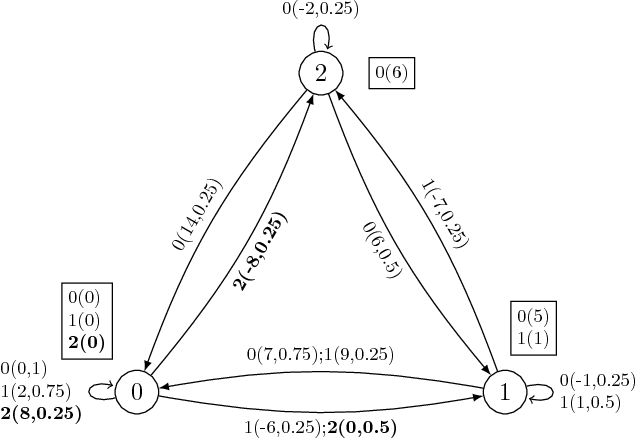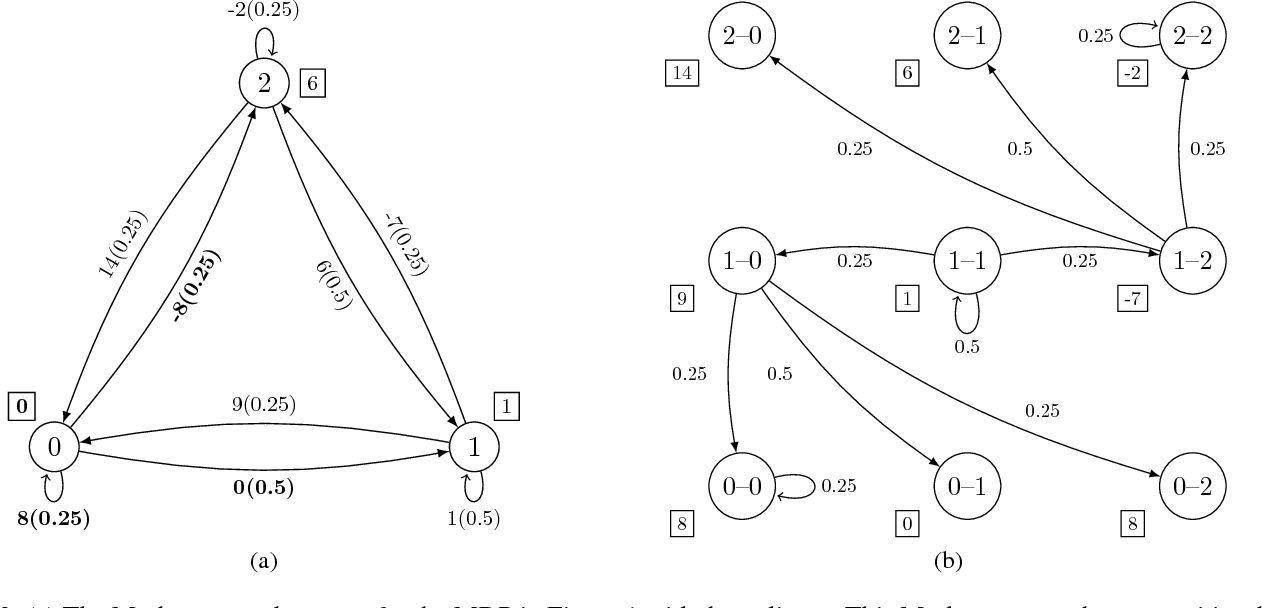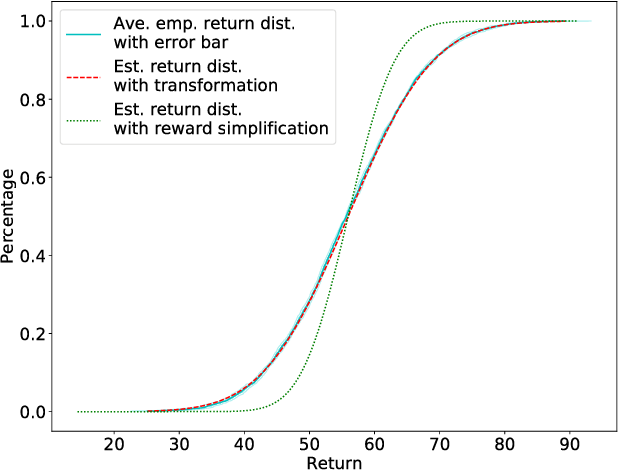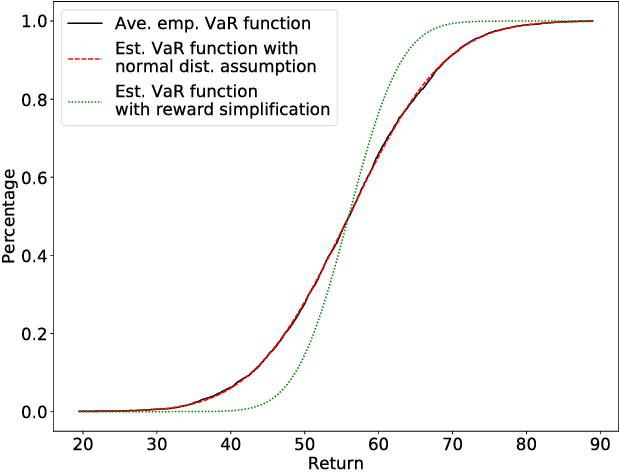Although the general deterministic reward function in MDPs takes three arguments - current state, action, and next state; it is often simplified to a function of two arguments - current state and action. The former is called a transition-based reward function, whereas the latter is called a state-based reward function. When the objective is a function of the expected cumulative reward only, this simplification works perfectly. However, when the objective is risk-sensitive - e.g., depends on the reward distribution, this simplification leads to incorrect values of the objective. This paper studies the distribution estimation of the cumulative discounted reward in infinite-horizon MDPs with finite state and action spaces. First, by taking the Value-at-Risk (VaR) objective as an example, we illustrate and analyze the error from the above simplification on the reward distribution. Next, we propose a transformation for MDPs to preserve the reward distribution and convert transition-based reward functions to deterministic state-based reward functions. This transformation works whether the transition-based reward function is deterministic or stochastic. Lastly, we show how to estimate the reward distribution after applying the proposed transformation in different settings, provided that the distribution is approximately normal.

Click to Read Paper and Get Code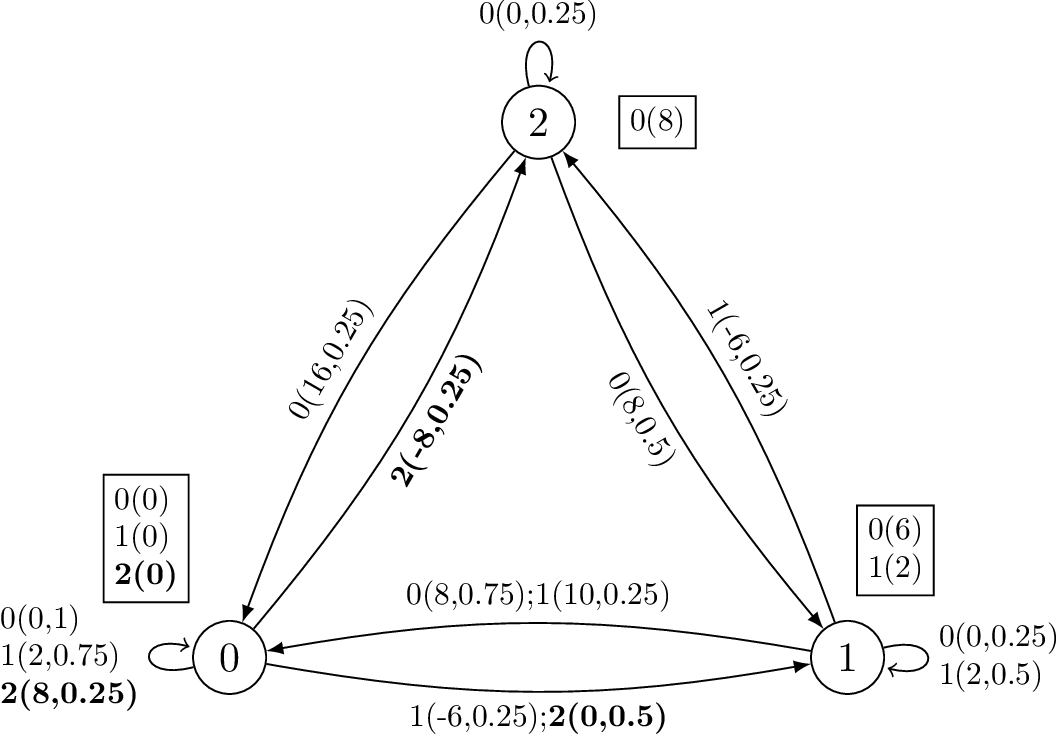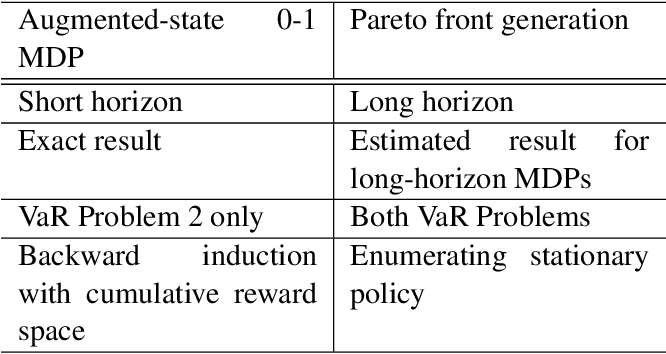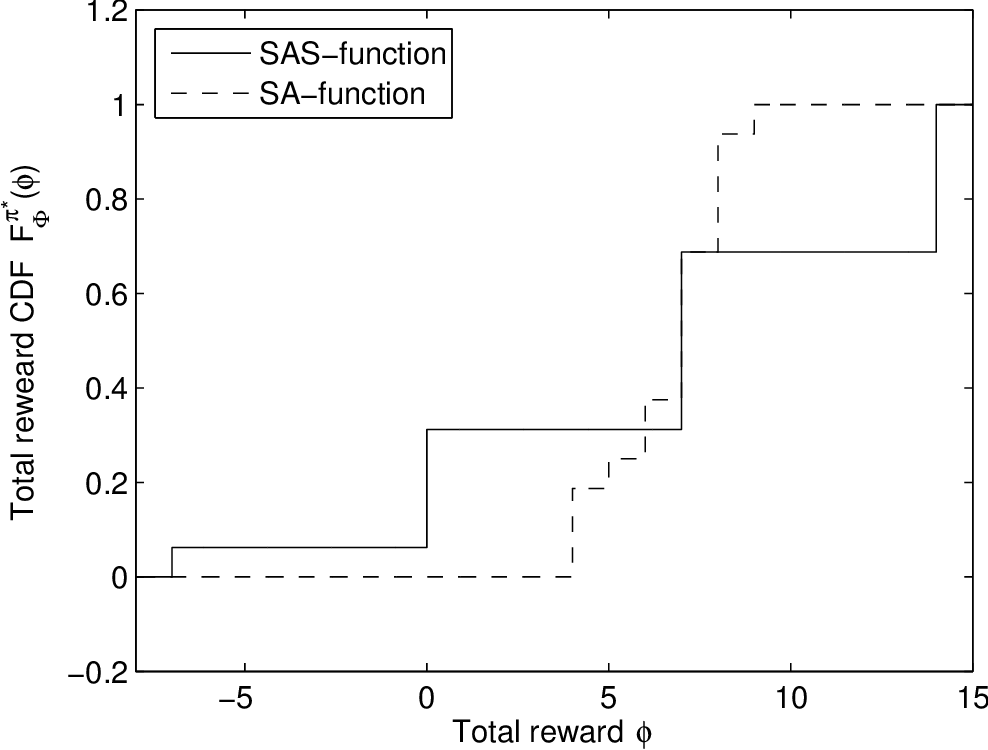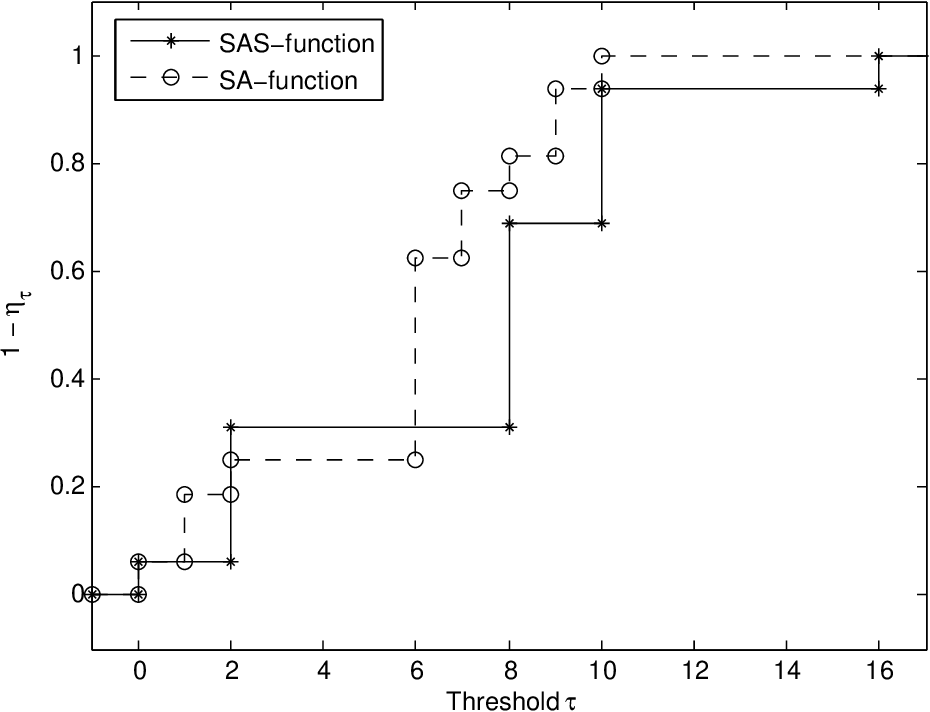This paper studies Value-at-Risk (VaR) problems in short- and long-horizon Markov decision processes (MDPs) with finite state space and two different reward functions. Firstly we examine the effects of two reward functions under two criteria in a short-horizon MDP. We show that under the VaR criterion, when the original reward function is on both current and next states, the reward simplification will change the VaR. Secondly, for long-horizon MDPs, we estimate the Pareto front of the total reward distribution set with the aid of spectral theory and the central limit theorem. Since the estimation is for a Markov process with the simplified reward function only, we present a transformation algorithm for the Markov process with the original reward function, in order to estimate the Pareto front with an intact total reward distribution.

* 23 pages, 5 figures
Click to Read Paper and Get Code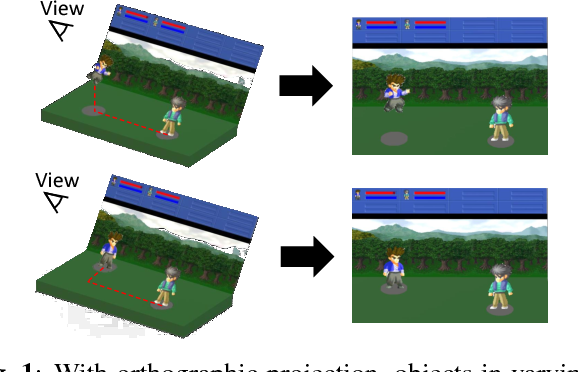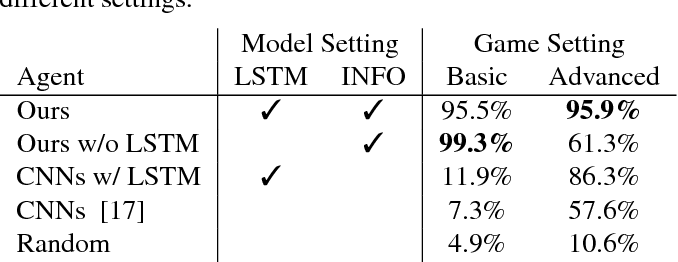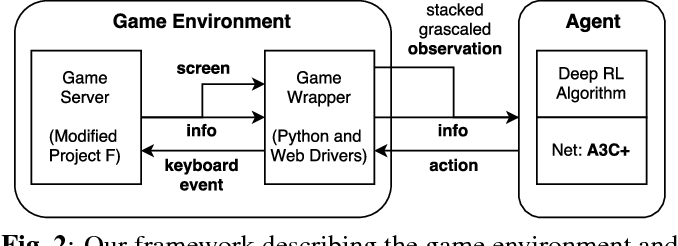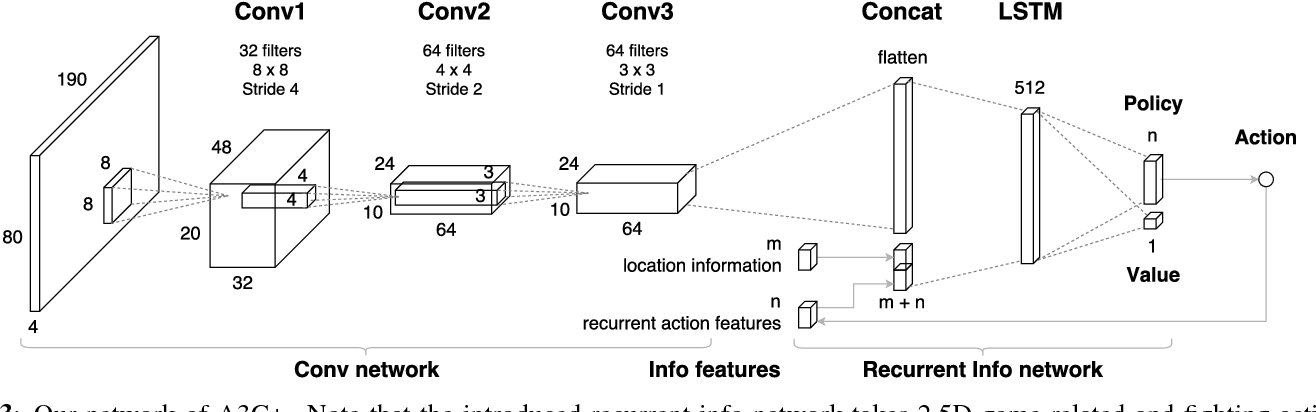Deep reinforcement learning has shown its success in game playing. However, 2.5D fighting games would be a challenging task to handle due to ambiguity in visual appearances like height or depth of the characters. Moreover, actions in such games typically involve particular sequential action orders, which also makes the network design very difficult. Based on the network of Asynchronous Advantage Actor-Critic (A3C), we create an OpenAI-gym-like gaming environment with the game of Little Fighter 2 (LF2), and present a novel A3C+ network for learning RL agents. The introduced model includes a Recurrent Info network, which utilizes game-related info features with recurrent layers to observe combo skills for fighting. In the experiments, we consider LF2 in different settings, which successfully demonstrates the use of our proposed model for learning 2.5D fighting games.

* ICIP 2018
Click to Read Paper and Get Code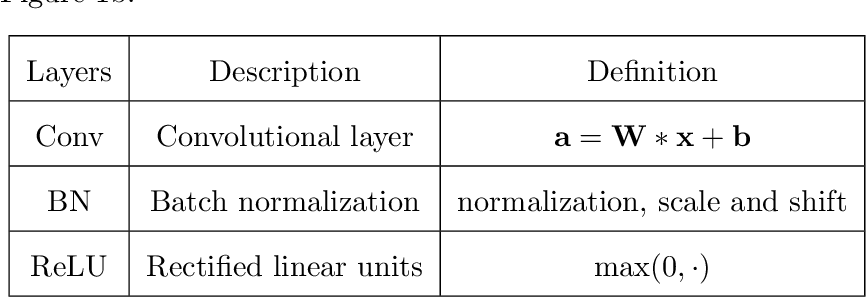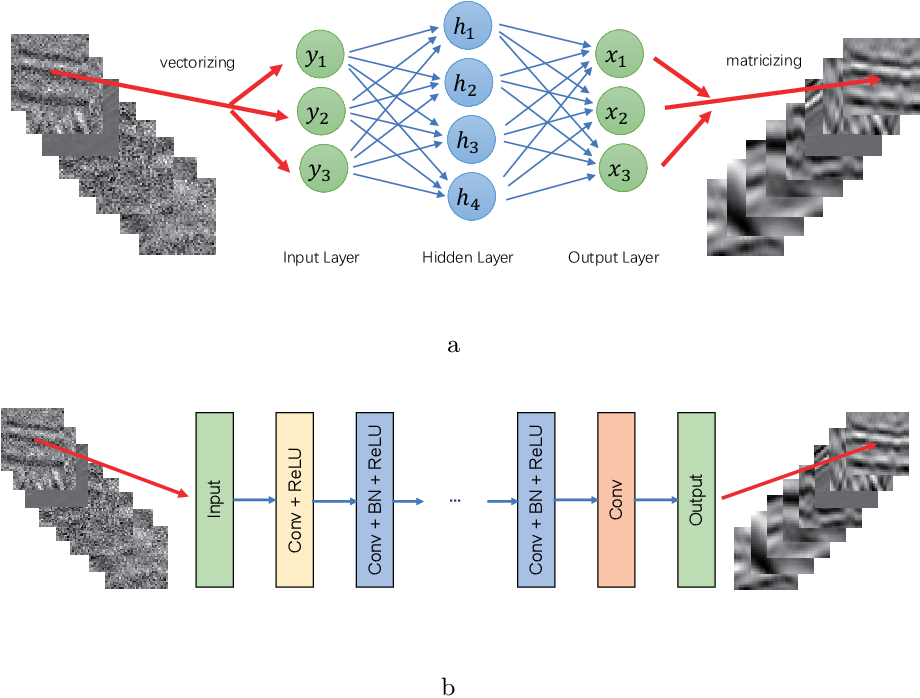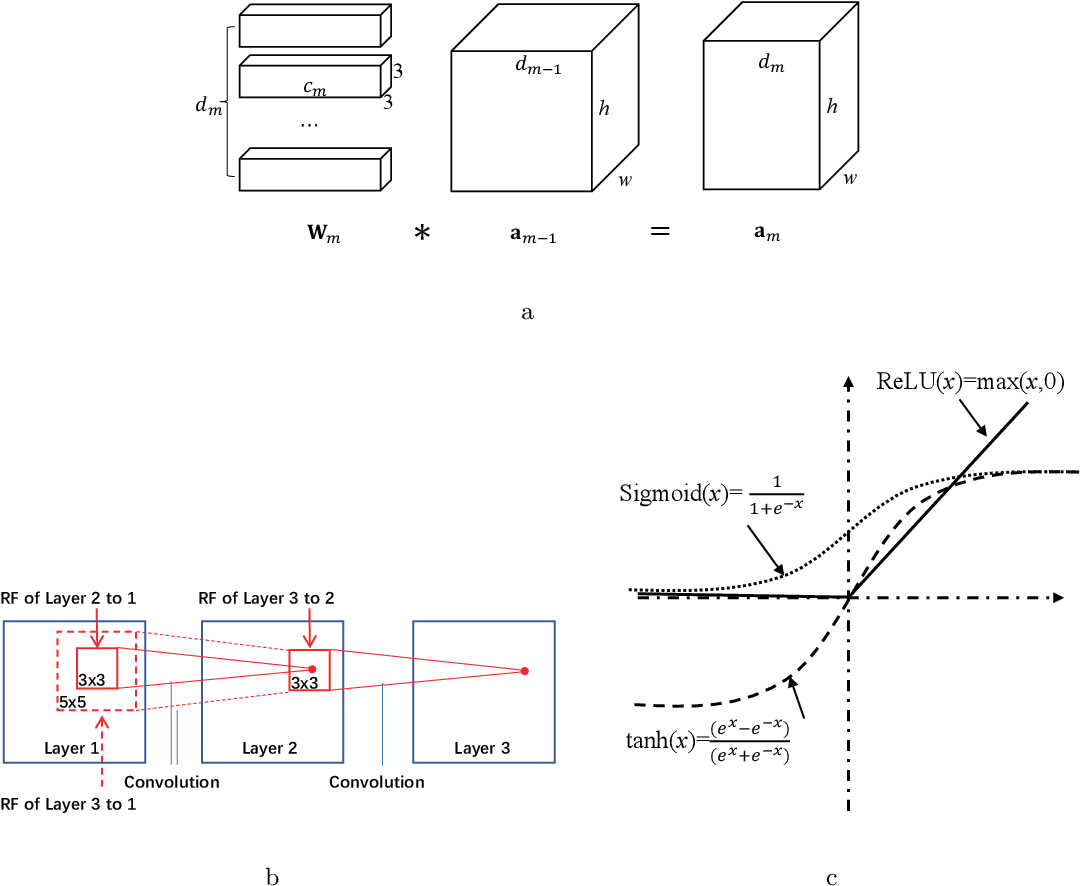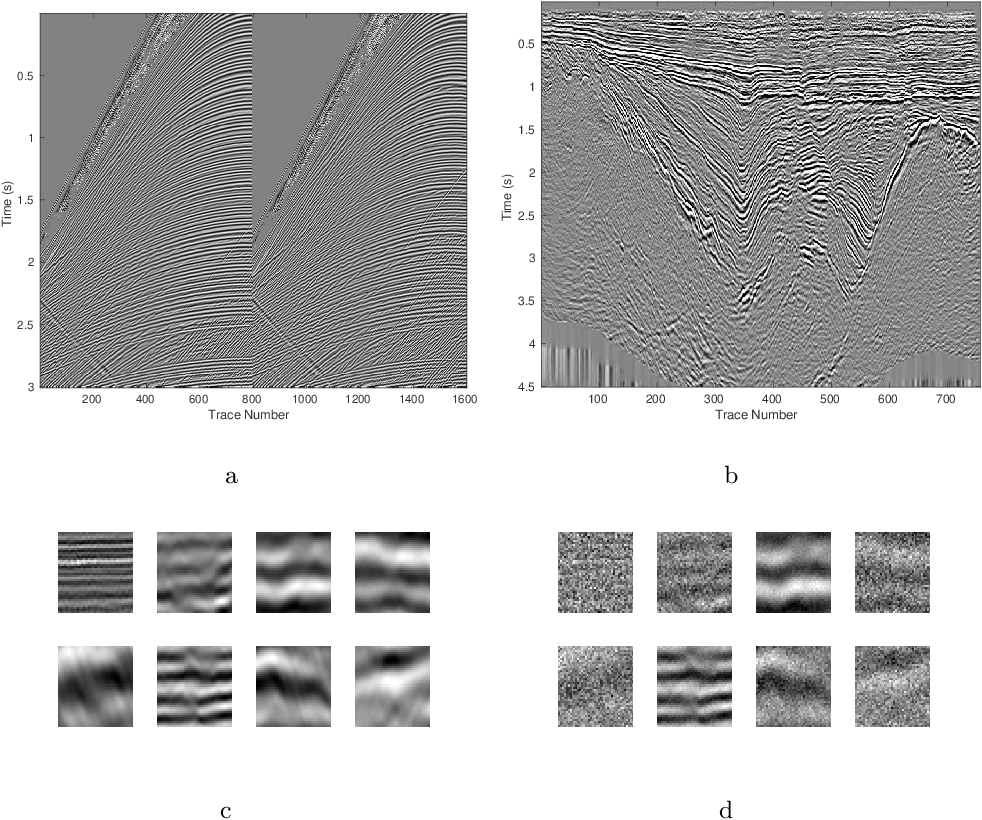We herein introduce deep learning to seismic noise attenuation. Compared with traditional seismic noise attenuation algorithms that depend on signal models and their corresponding prior assumptions, a deep neural network is trained based on a large training set, where the inputs are the raw datasets and the corresponding outputs are the desired clean data. After the completion of training, the deep learning method achieves adaptive denoising with no requirements of (i) accurate modeling of the signal and noise, and (ii) optimal parameters tuning. We call this intelligent denoising. We use a convolutional neural network as the basic tool for deep learning. The training set is generated with manually added noise in random and linear noise attenuation, and with the wave equation in the multiple attenuation. Stochastic gradient descent is used to solve the optimal parameters for the convolutional neural network. The runtime of deep learning on a graphics processing unit for denoising has the same order as the $f-x$ deconvolutional method. Synthetic and field results show the potential applications of deep learning in the automation of random noise attenuation with unknown variance, linear noise, and multiples.

* 54 pages, 34 figures
Click to Read Paper and Get Code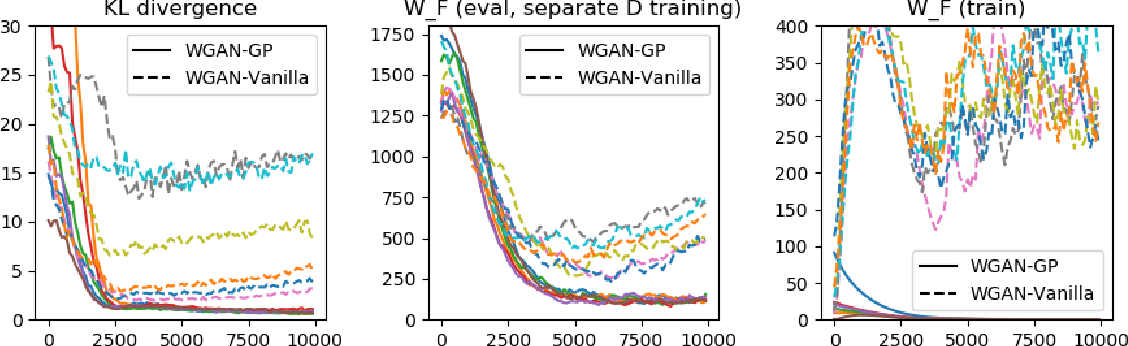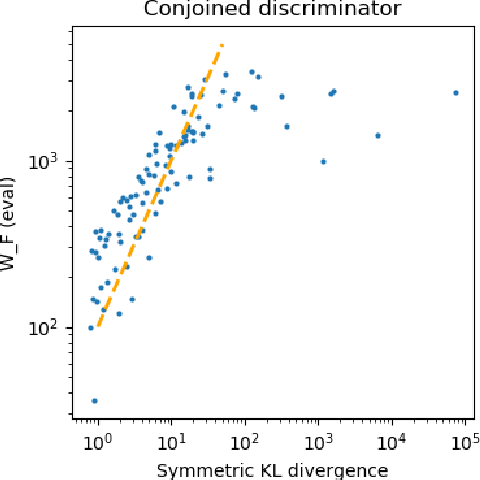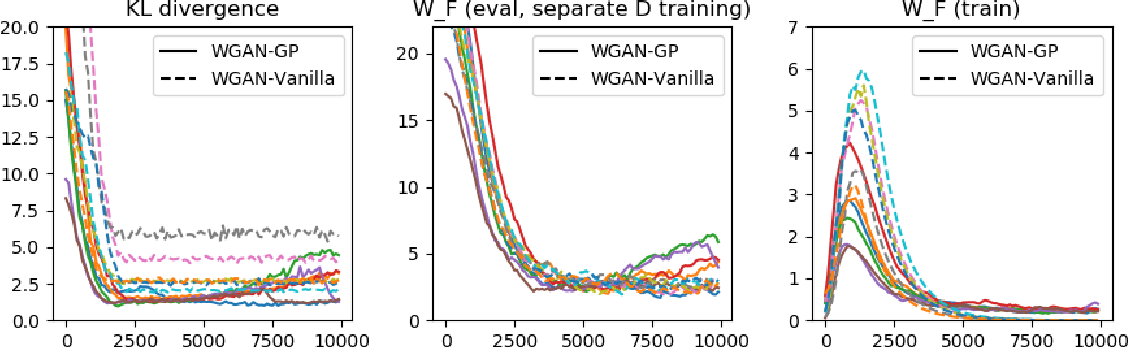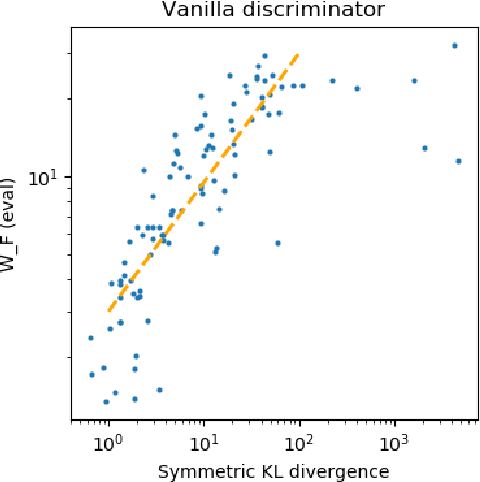While Generative Adversarial Networks (GANs) have empirically produced impressive results on learning complex real-world distributions, recent work has shown that they suffer from lack of diversity or mode collapse. The theoretical work of Arora et al. suggests a dilemma about GANs' statistical properties: powerful discriminators cause overfitting, whereas weak discriminators cannot detect mode collapse. In contrast, we show in this paper that GANs can in principle learn distributions in Wasserstein distance (or KL-divergence in many cases) with polynomial sample complexity, if the discriminator class has strong distinguishing power against the particular generator class (instead of against all possible generators). For various generator classes such as mixture of Gaussians, exponential families, and invertible neural networks generators, we design corresponding discriminators (which are often neural nets of specific architectures) such that the Integral Probability Metric (IPM) induced by the discriminators can provably approximate the Wasserstein distance and/or KL-divergence. This implies that if the training is successful, then the learned distribution is close to the true distribution in Wasserstein distance or KL divergence, and thus cannot drop modes. Our preliminary experiments show that on synthetic datasets the test IPM is well correlated with KL divergence, indicating that the lack of diversity may be caused by the sub-optimality in optimization instead of statistical inefficiency.

Click to Read Paper and Get Code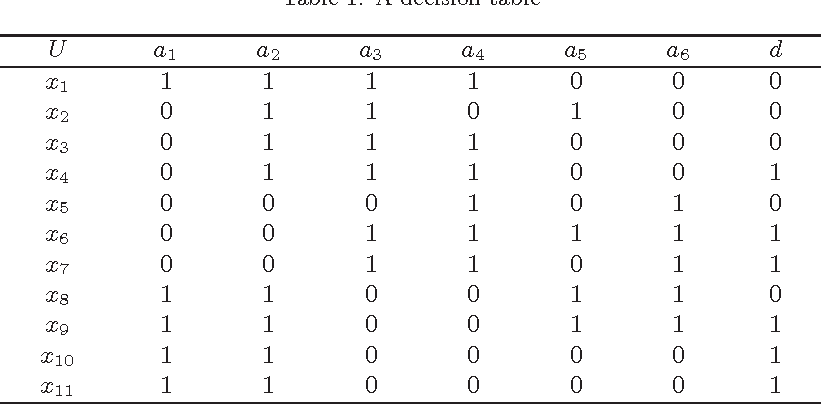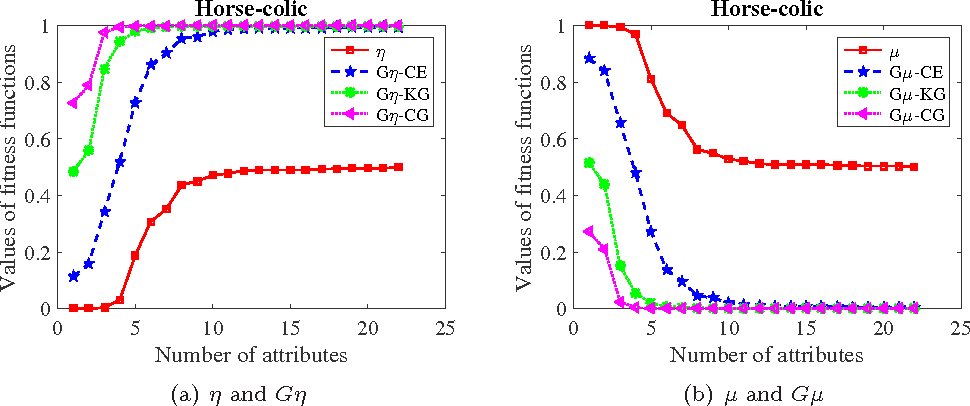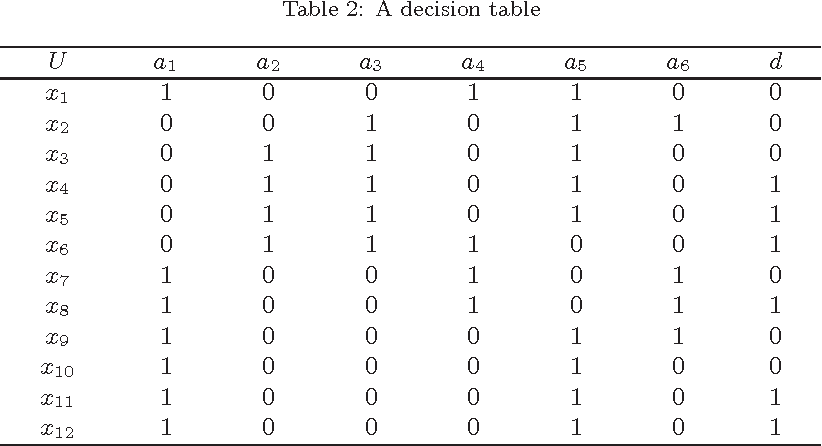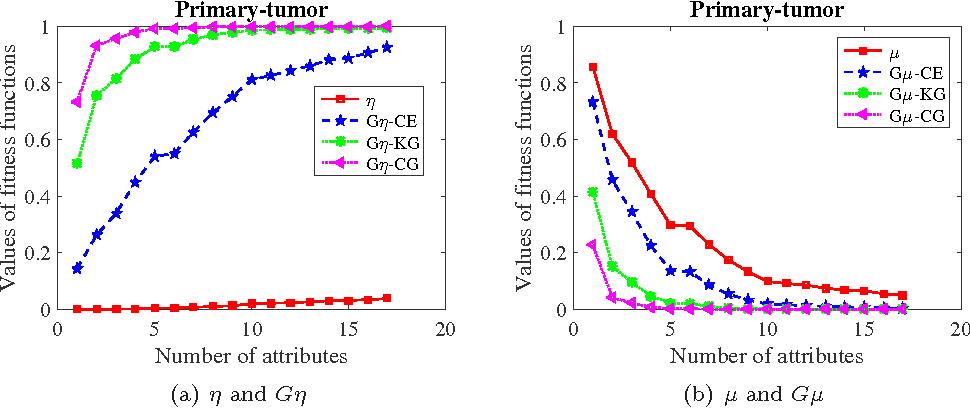Attribute reduction is one of the most important topics in rough set theory. Heuristic attribute reduction algorithms have been presented to solve the attribute reduction problem. It is generally known that fitness functions play a key role in developing heuristic attribute reduction algorithms. The monotonicity of fitness functions can guarantee the validity of heuristic attribute reduction algorithms. In probabilistic rough set model, distribution reducts can ensure the decision rules derived from the reducts are compatible with those derived from the original decision table. However, there are few studies on developing heuristic attribute reduction algorithms for finding distribution reducts. This is partly due to the fact that there are no monotonic fitness functions that are used to design heuristic attribute reduction algorithms in probabilistic rough set model. The main objective of this paper is to develop heuristic attribute reduction algorithms for finding distribution reducts in probabilistic rough set model. For one thing, two monotonic fitness functions are constructed, from which equivalence definitions of distribution reducts can be obtained. For another, two modified monotonic fitness functions are proposed to evaluate the significance of attributes more effectively. On this basis, two heuristic attribute reduction algorithms for finding distribution reducts are developed based on addition-deletion method and deletion method. In particular, the monotonicity of fitness functions guarantees the rationality of the proposed heuristic attribute reduction algorithms. Results of experimental analysis are included to quantify the effectiveness of the proposed fitness functions and distribution reducts.

* 44 pages, 24 figures
Click to Read Paper and Get Code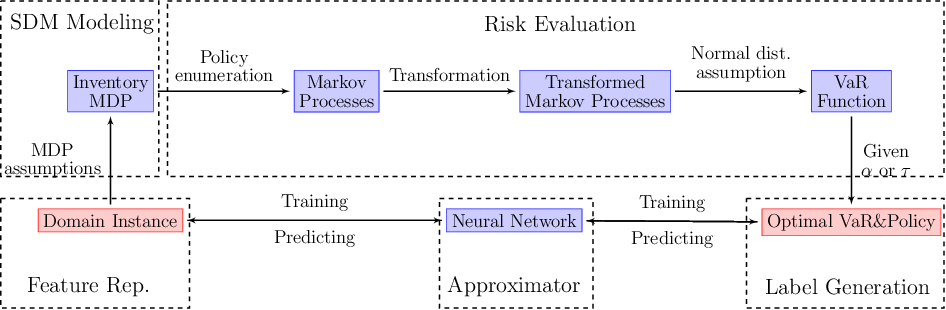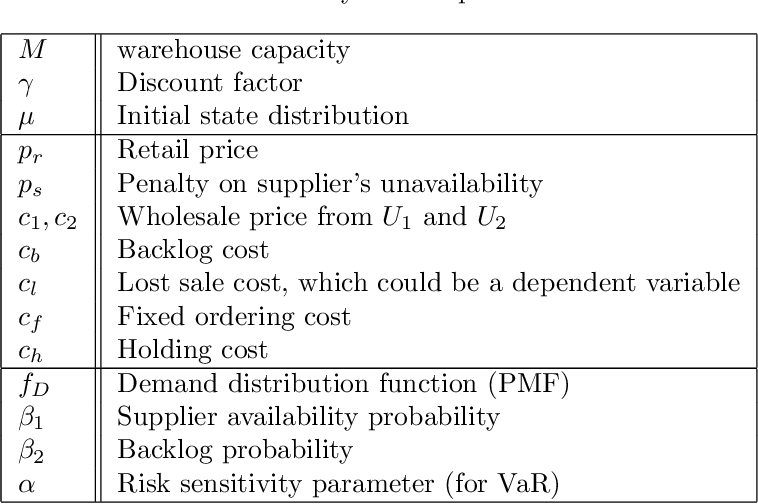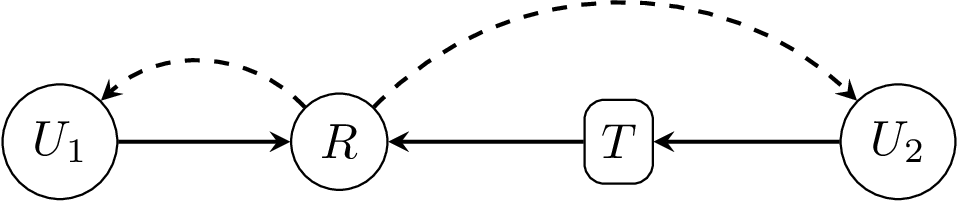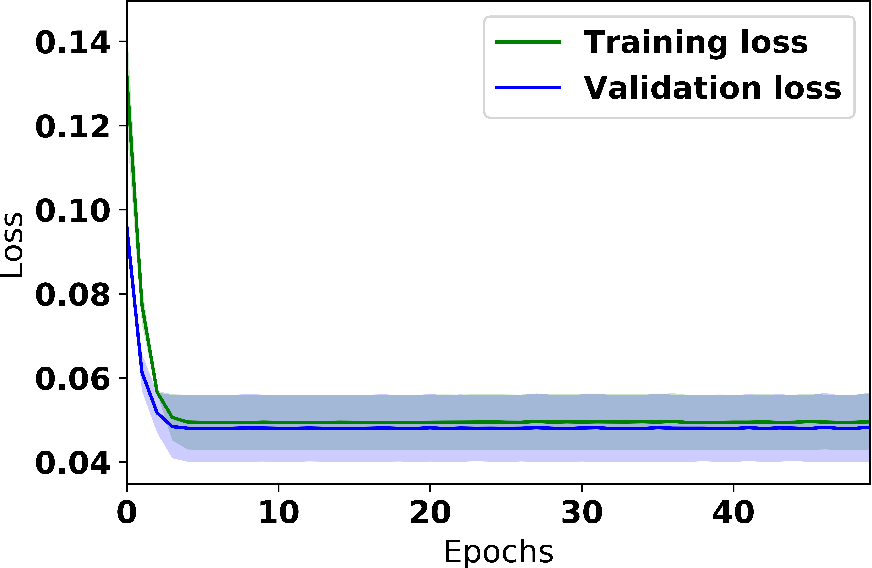We present a scheme for sequential decision making with a risk-sensitive objective and constraints in a dynamic environment. A neural network is trained as an approximator of the mapping from parameter space to space of risk and policy with risk-sensitive constraints. For a given risk-sensitive problem, in which the objective and constraints are, or can be estimated by, functions of the mean and variance of return, we generate a synthetic dataset as training data. Parameters defining a targeted process might be dynamic, i.e., they might vary over time, so we sample them within specified intervals to deal with these dynamics. We show that: i). Most risk measures can be estimated using return variance; ii). By virtue of the state-augmentation transformation, practical problems modeled by Markov decision processes with stochastic rewards can be solved in a risk-sensitive scenario; and iii). The proposed scheme is validated by a numerical experiment.

* 20 pages, 3 figures
Click to Read Paper and Get Code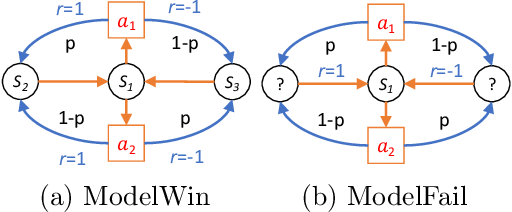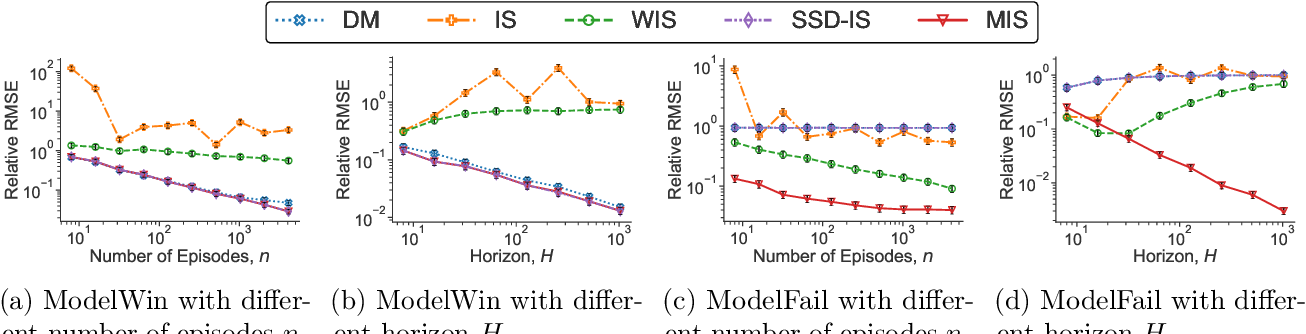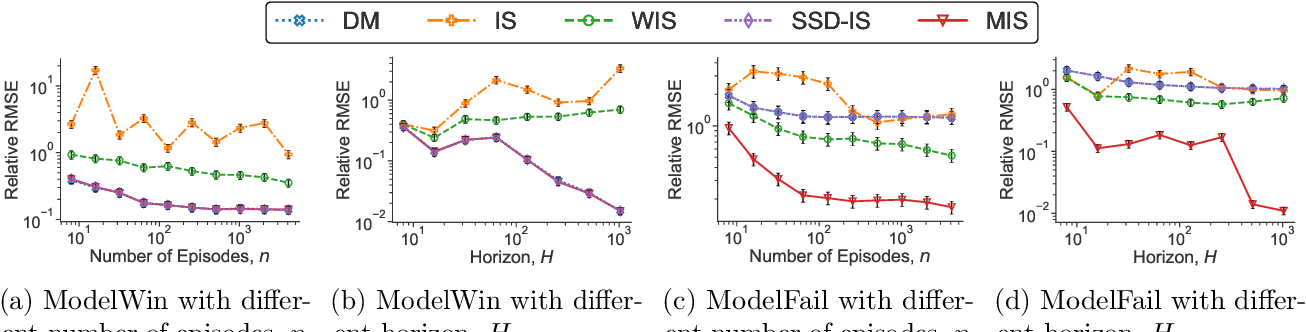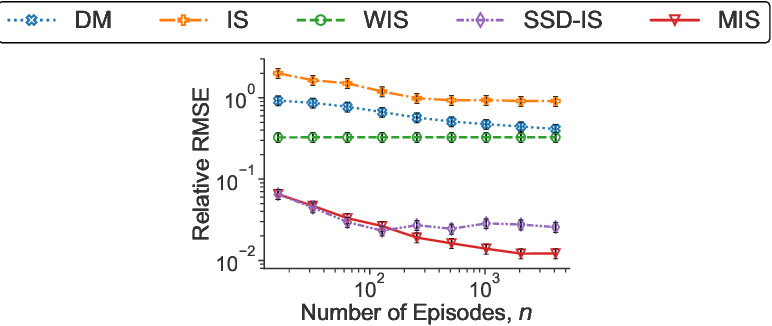Motivated by the many real-world applications of reinforcement learning (RL) that require safe-policy iterations, we consider the problem of off-policy evaluation (OPE) --- the problem of evaluating a new policy using the historical data obtained by different behavior policies --- under the model of nonstationary episodic Markov Decision Processes with a long horizon and large action space. Existing importance sampling (IS) methods often suffer from large variance that depends exponentially on the RL horizon $H$. To solve this problem, we consider a marginalized importance sampling (MIS) estimator that recursively estimates the state marginal distribution for the target policy at every step. MIS achieves a mean-squared error of $O(H^2R_{\max}^2\sum_{t=1}^H\mathbb E_\mu[(w_{\pi,\mu}(s_t,a_t))^2]/n)$ for large $n$, where $w_{\pi,\mu}(s_t,a_t)$ is the ratio of the marginal distribution of $t$th step under $\pi$ and $\mu$, $H$ is the horizon, $R_{\max}$ is the maximal rewards, and $n$ is the sample size. The result nearly matches the Cramer-Rao lower bounds for DAG MDP in \citet{jiang2016doubly} for most non-trivial regimes. To the best of our knowledge, this is the first OPE estimator with provably optimal dependence in $H$ and the second moments of the importance weight. Besides theoretical optimality, we empirically demonstrate the superiority of our method in time-varying, partially observable, and long-horizon RL environments.

Click to Read Paper and Get Code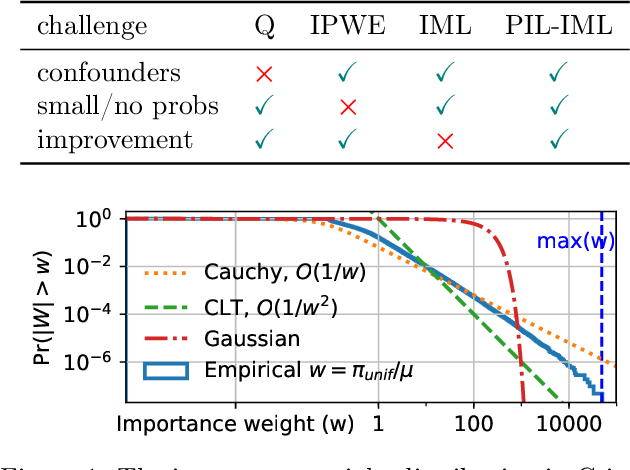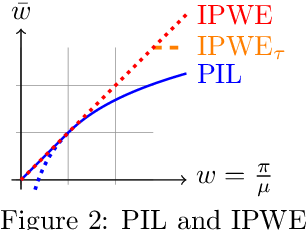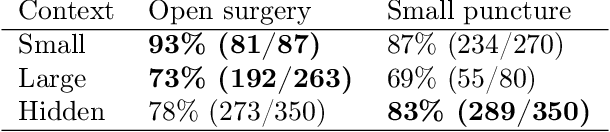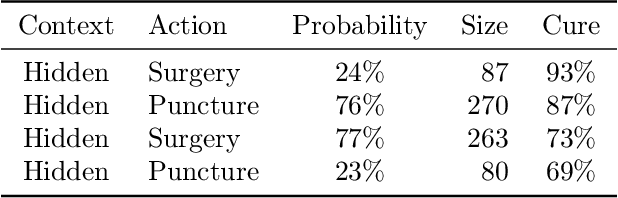We study the problem of offline learning in automated decision systems under the contextual bandits model. We are given logged historical data consisting of contexts, (randomized) actions, and (nonnegative) rewards. A common goal is to evaluate what would happen if different actions were taken in the same contexts, so as to optimize the action policies accordingly. The typical approach to this problem, inverse probability weighted estimation (IPWE) [Bottou et al., 2013], requires logged action probabilities, which may be missing in practice due to engineering complications. Even when available, small action probabilities cause large uncertainty in IPWE, rendering the corresponding results insignificant. To solve both problems, we show how one can use policy improvement (PIL) objectives, regularized by policy imitation (IML). We motivate and analyze PIL as an extension to Clipped-IPWE, by showing that both are lower-bound surrogates to the vanilla IPWE. We also formally connect IML to IPWE variance estimation [Swaminathan and Joachims 2015] and natural policy gradients. Without probability logging, our PIL-IML interpretations justify and improve, by reward-weighting, the state-of-art cross-entropy (CE) loss that predicts the action items among all action candidates available in the same contexts. With probability logging, our main theoretical contribution connects IML-underfitting to the existence of either confounding variables or model misspecification. We show the value and accuracy of our insights by simulations based on Simpson's paradox, standard UCI multiclass-to-bandit conversions and on the Criteo counterfactual analysis challenge dataset.

* Accepted for publication at AISTATS 2019
Click to Read Paper and Get Code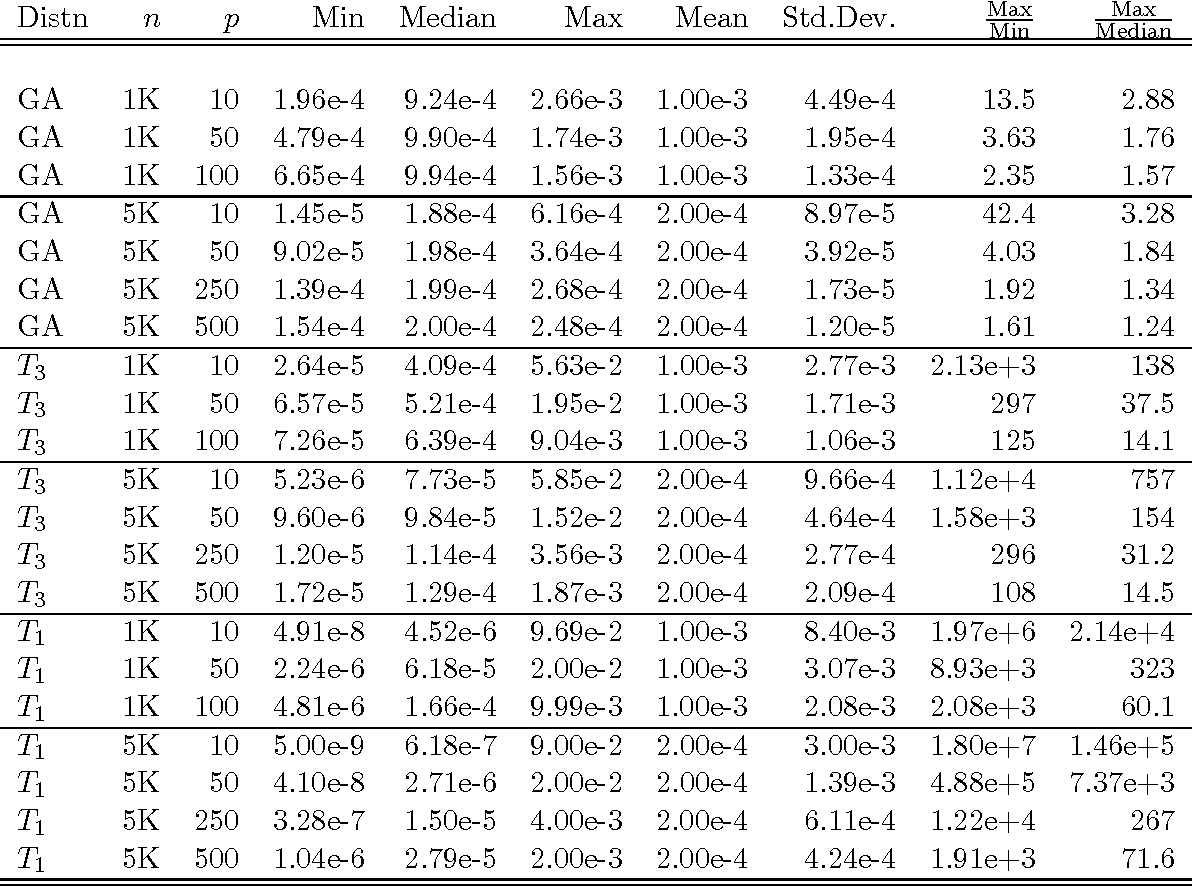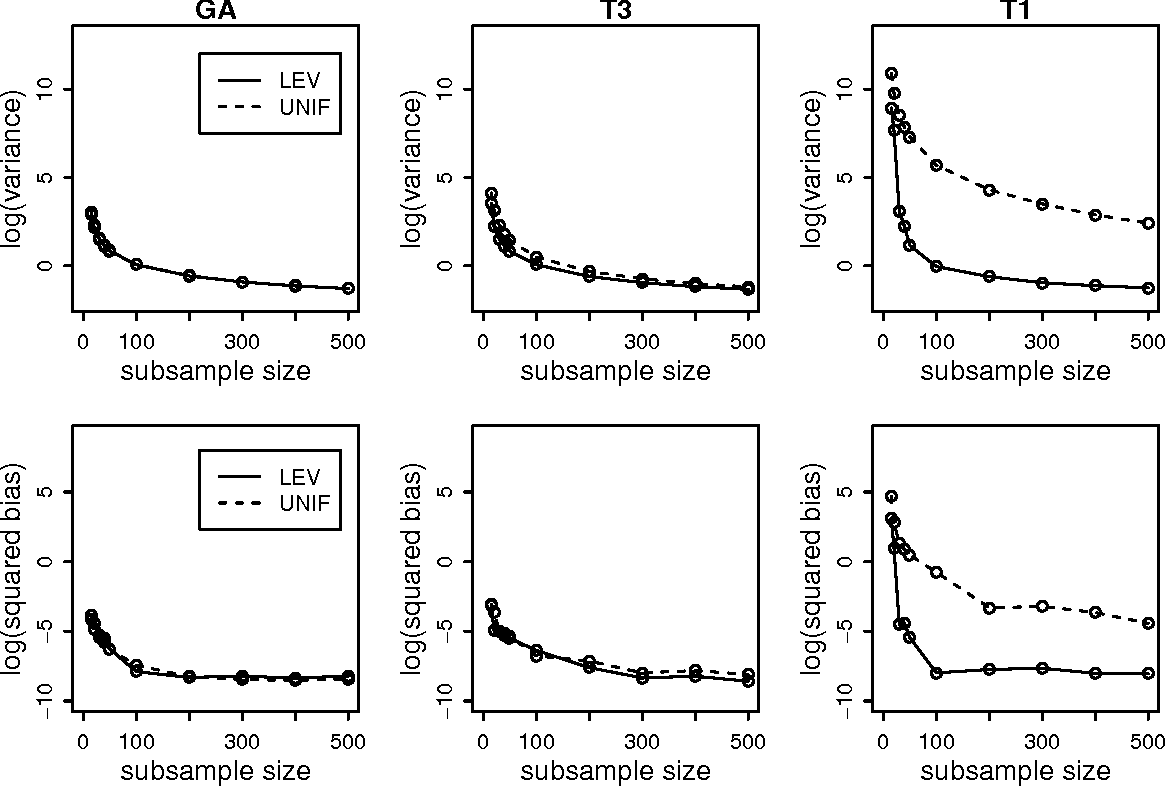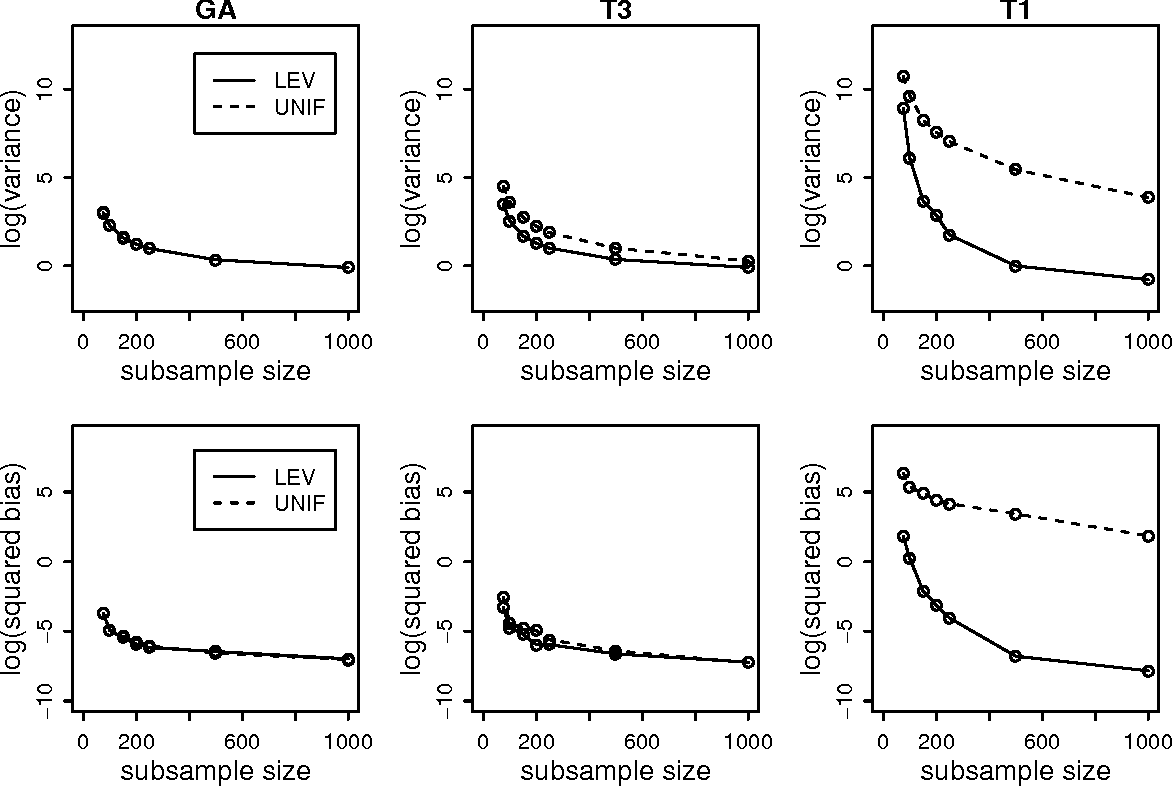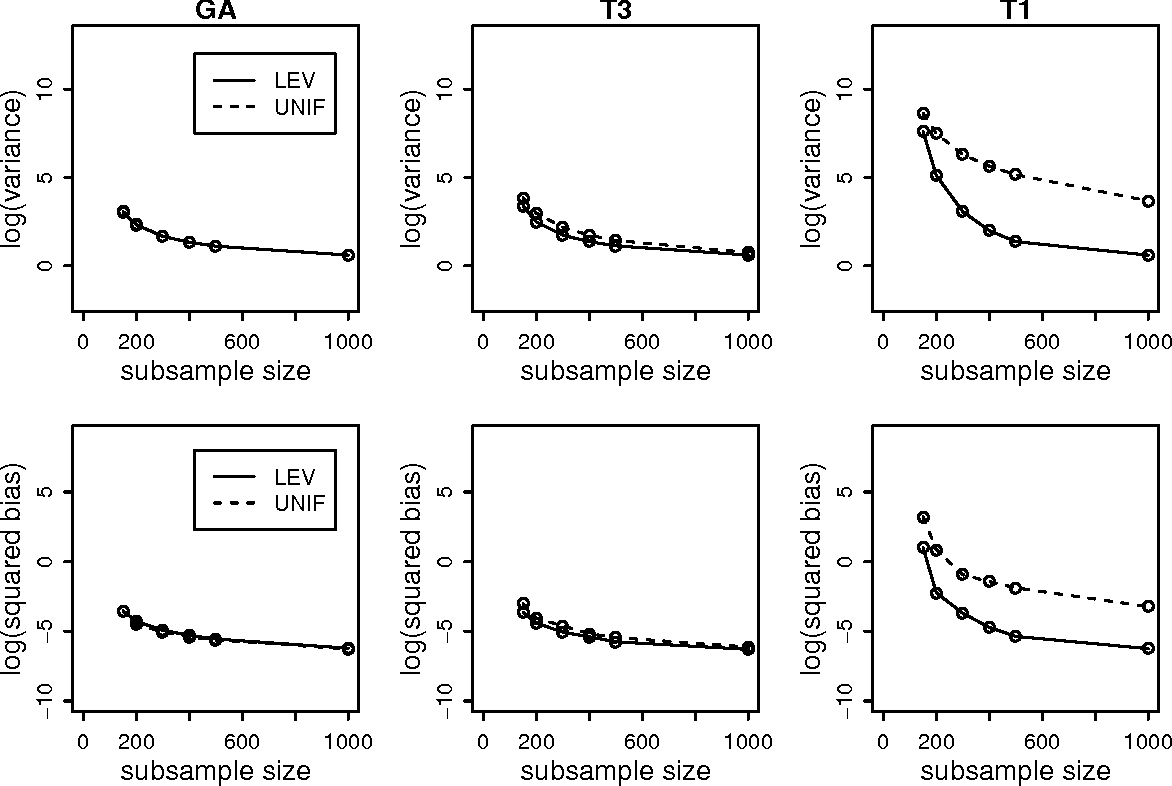One popular method for dealing with large-scale data sets is sampling. For example, by using the empirical statistical leverage scores as an importance sampling distribution, the method of algorithmic leveraging samples and rescales rows/columns of data matrices to reduce the data size before performing computations on the subproblem. This method has been successful in improving computational efficiency of algorithms for matrix problems such as least-squares approximation, least absolute deviations approximation, and low-rank matrix approximation. Existing work has focused on algorithmic issues such as worst-case running times and numerical issues associated with providing high-quality implementations, but none of it addresses statistical aspects of this method. In this paper, we provide a simple yet effective framework to evaluate the statistical properties of algorithmic leveraging in the context of estimating parameters in a linear regression model with a fixed number of predictors. We show that from the statistical perspective of bias and variance, neither leverage-based sampling nor uniform sampling dominates the other. This result is particularly striking, given the well-known result that, from the algorithmic perspective of worst-case analysis, leverage-based sampling provides uniformly superior worst-case algorithmic results, when compared with uniform sampling. Based on these theoretical results, we propose and analyze two new leveraging algorithms. A detailed empirical evaluation of existing leverage-based methods as well as these two new methods is carried out on both synthetic and real data sets. The empirical results indicate that our theory is a good predictor of practical performance of existing and new leverage-based algorithms and that the new algorithms achieve improved performance.

* 44 pages, 17 figures
Click to Read Paper and Get Code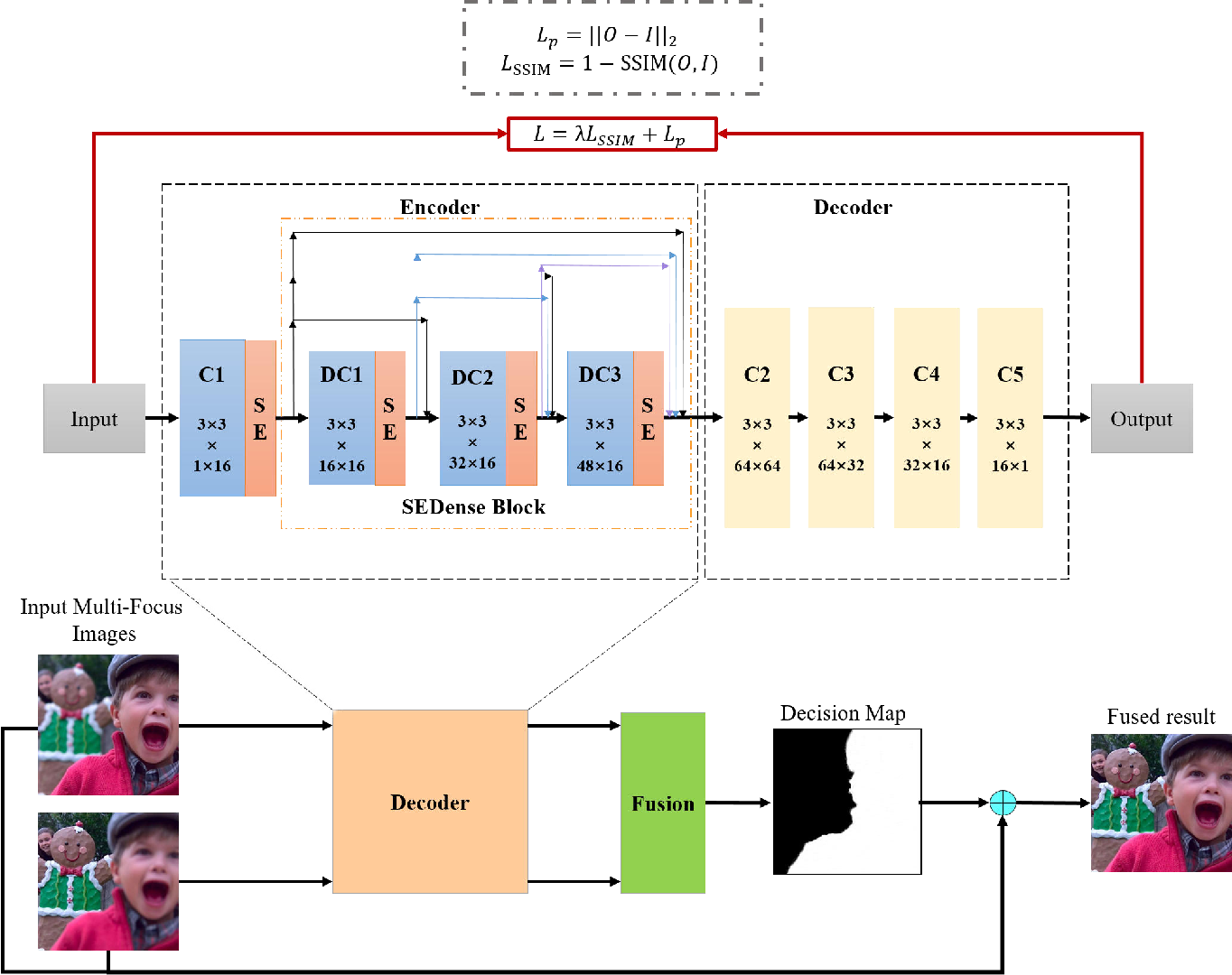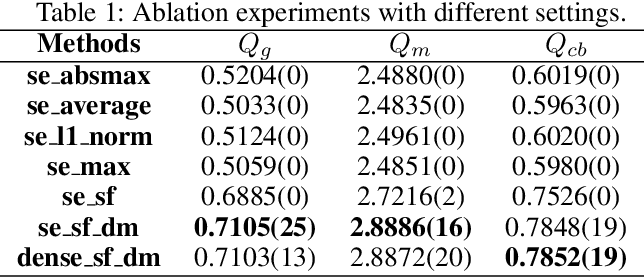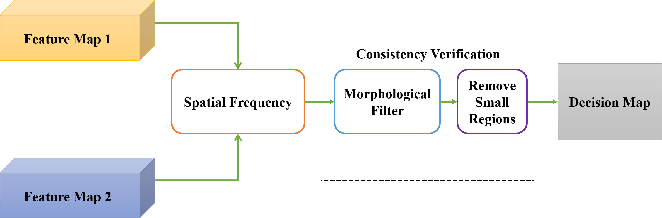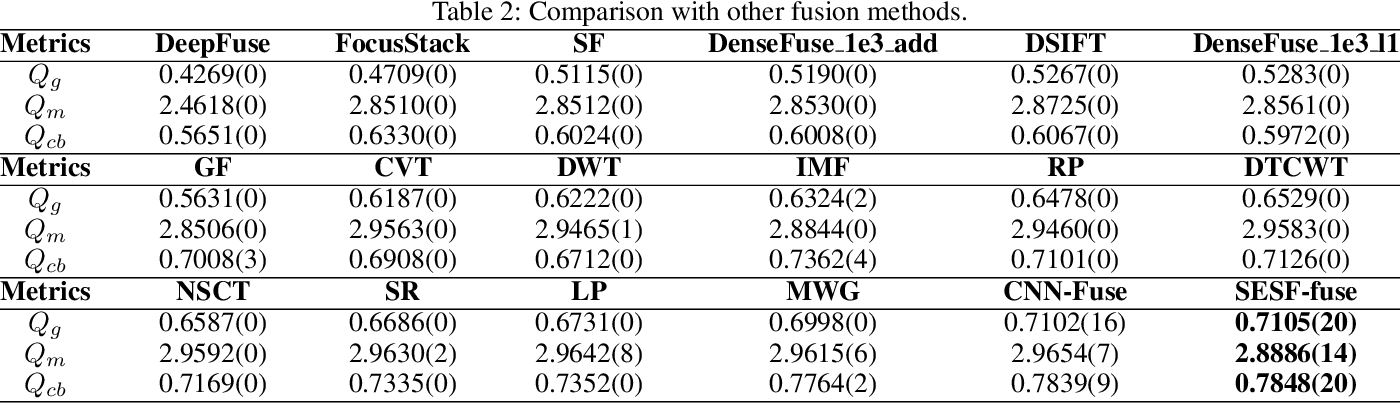In this work, we propose a novel unsupervised deep learning model to address multi-focus image fusion problem. First, we train an encoder-decoder network in unsupervised manner to acquire deep feature of input images. And then we utilize these features and spatial frequency to measure activity level and decision map. Finally, we apply some consistency verification methods to adjust the decision map and draw out fused result. The key point behind of proposed method is that only the objects within the depth-of-field (DOF) have sharp appearance in the photograph while other objects are likely to be blurred. In contrast to previous works, our method analyzes sharp appearance in deep feature instead of original image. Experimental results demonstrate that the proposed method achieves the state-of-art fusion performance compared to existing 16 fusion methods in objective and subjective assessment.

* technological report and fix some errors
Click to Read Paper and Get Code